library(ggstats)

The purpose of ggcoef_model() is to quickly plot the coefficients of a model. It is an updated and improved version of GGally::ggcoef() based on broom.helpers::tidy_plus_plus(). For displaying a nicely formatted table of the same models, look at gtsummary::tbl_regression().

## Quick coefficients plot

To work automatically, this function requires the broom.helpers. Simply call ggcoef_model() with a model object. It could be the result of stats::lm, stats::glm or any other model covered by broom.helpers.

data(tips, package = "reshape")
mod_simple <- lm(tip ~ day + time + total_bill, data = tips)
ggcoef_model(mod_simple)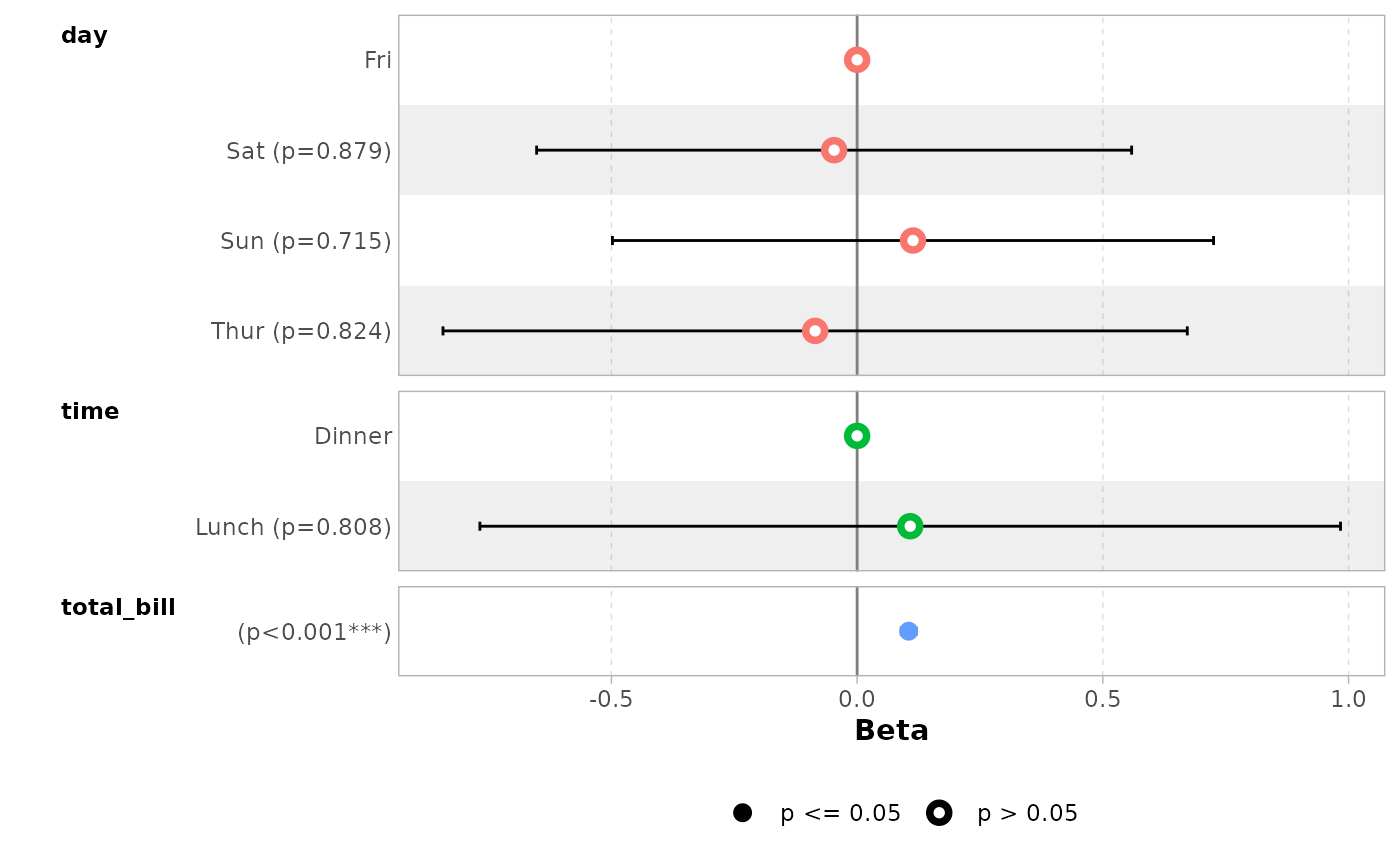In the case of a logistic regression (or any other model for which coefficients are usually exponentiated), simply indicated exponentiate = TRUE. Note that a logarithmic scale will be used for the x-axis.

d_titanic <- as.data.frame(Titanic)
d_titanic$Survived <- factor(d_titanic$Survived, c("No", "Yes"))
mod_titanic <- glm(
Survived ~ Sex * Age + Class,
weights = Freq,
data = d_titanic,
family = binomial
)
ggcoef_model(mod_titanic, exponentiate = TRUE)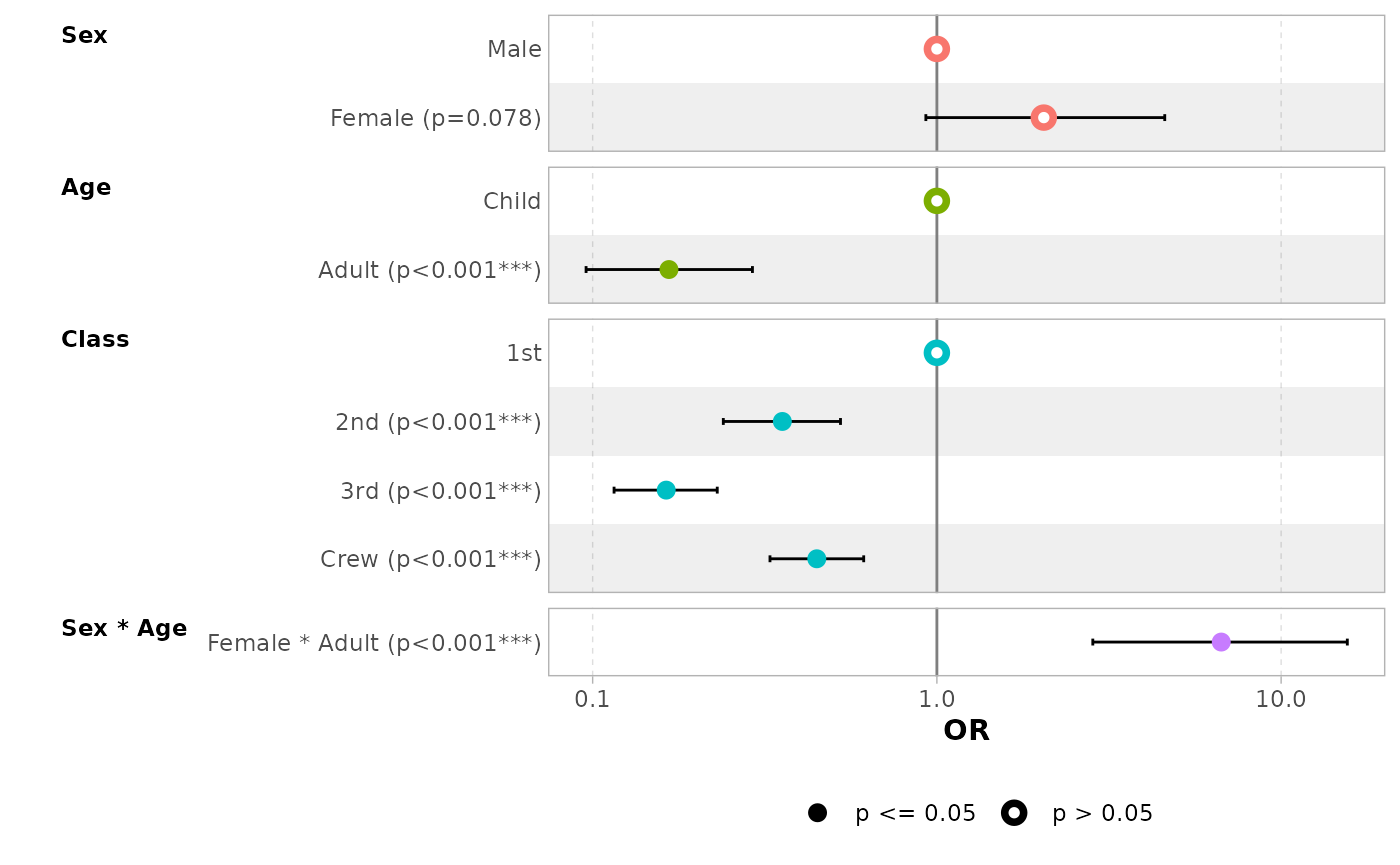## Customizing the plot

### Variable labels

You can use the labelled package to define variable labels. They will be automatically used by ggcoef_model(). Note that variable labels should be defined before computing the model.

library(labelled)
tips_labelled <- tips %>%
set_variable_labels(
day = "Day of the week",
time = "Lunch or Dinner",
total_bill = "Bill's total"
)
mod_labelled <- lm(tip ~ day + time + total_bill, data = tips_labelled)
ggcoef_model(mod_labelled)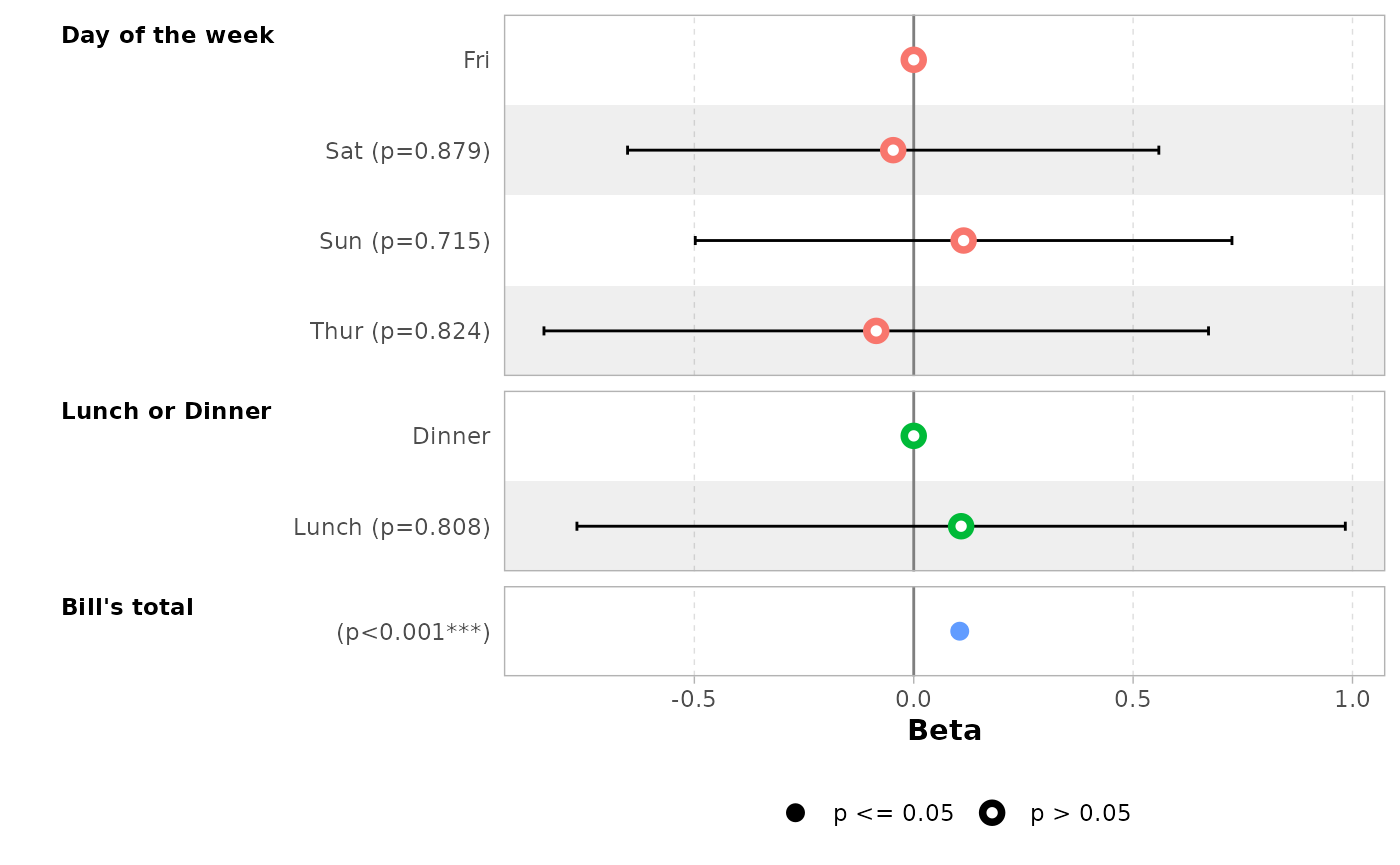You can also define custom variable labels directly by passing a named vector to the variable_labels option.

ggcoef_model(
mod_simple,
variable_labels = c(
day = "Week day",
time = "Time (lunch or dinner ?)",
total_bill = "Total of the bill"
)
)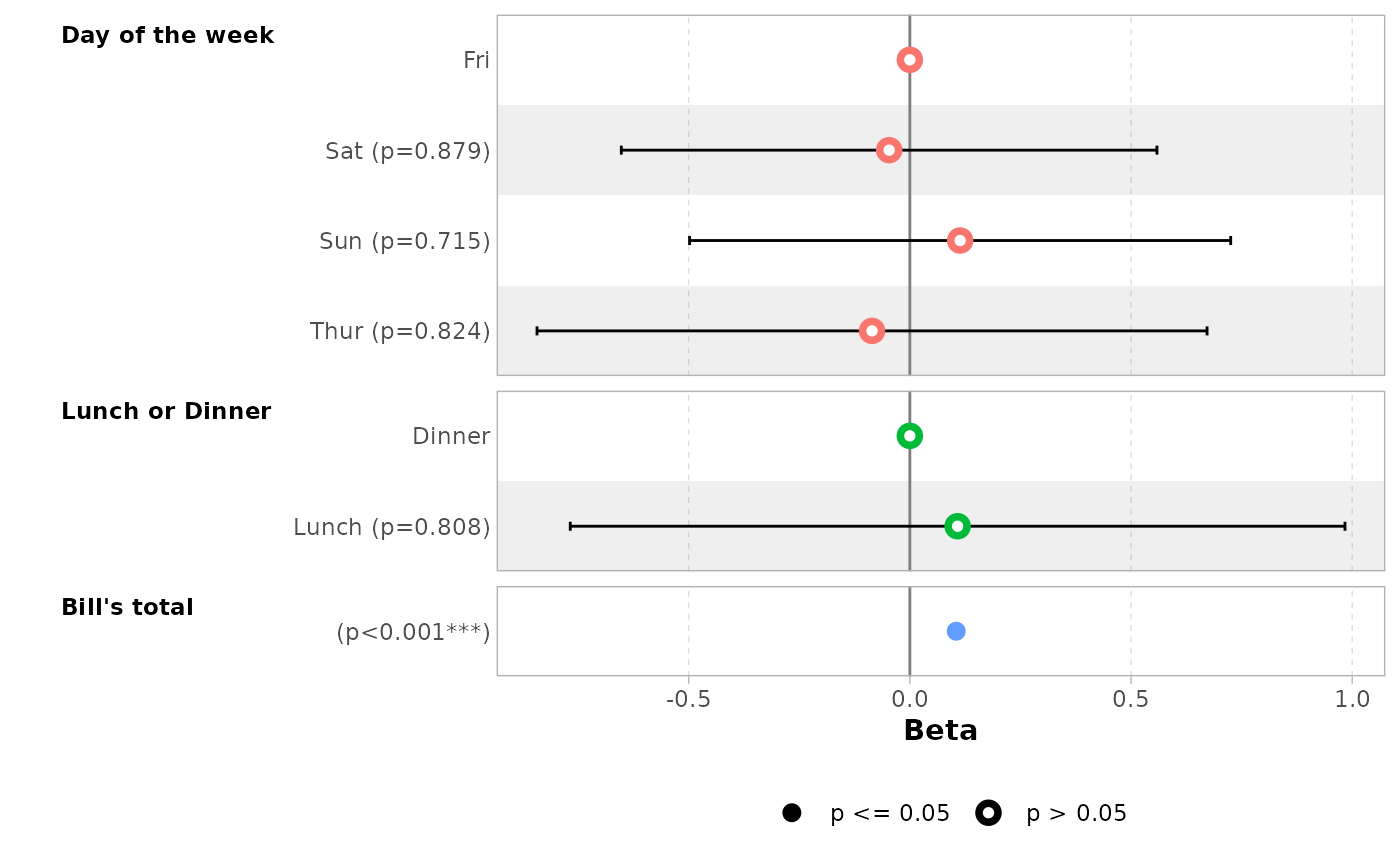If variable labels are to long, you can pass ggplot2::label_wrap_gen() or any other labeller function to facet_labeller.

ggcoef_model(
mod_simple,
variable_labels = c(
day = "Week day",
time = "Time (lunch or dinner ?)",
total_bill = "Total of the bill"
),
facet_labeller = ggplot2::label_wrap_gen(10)
)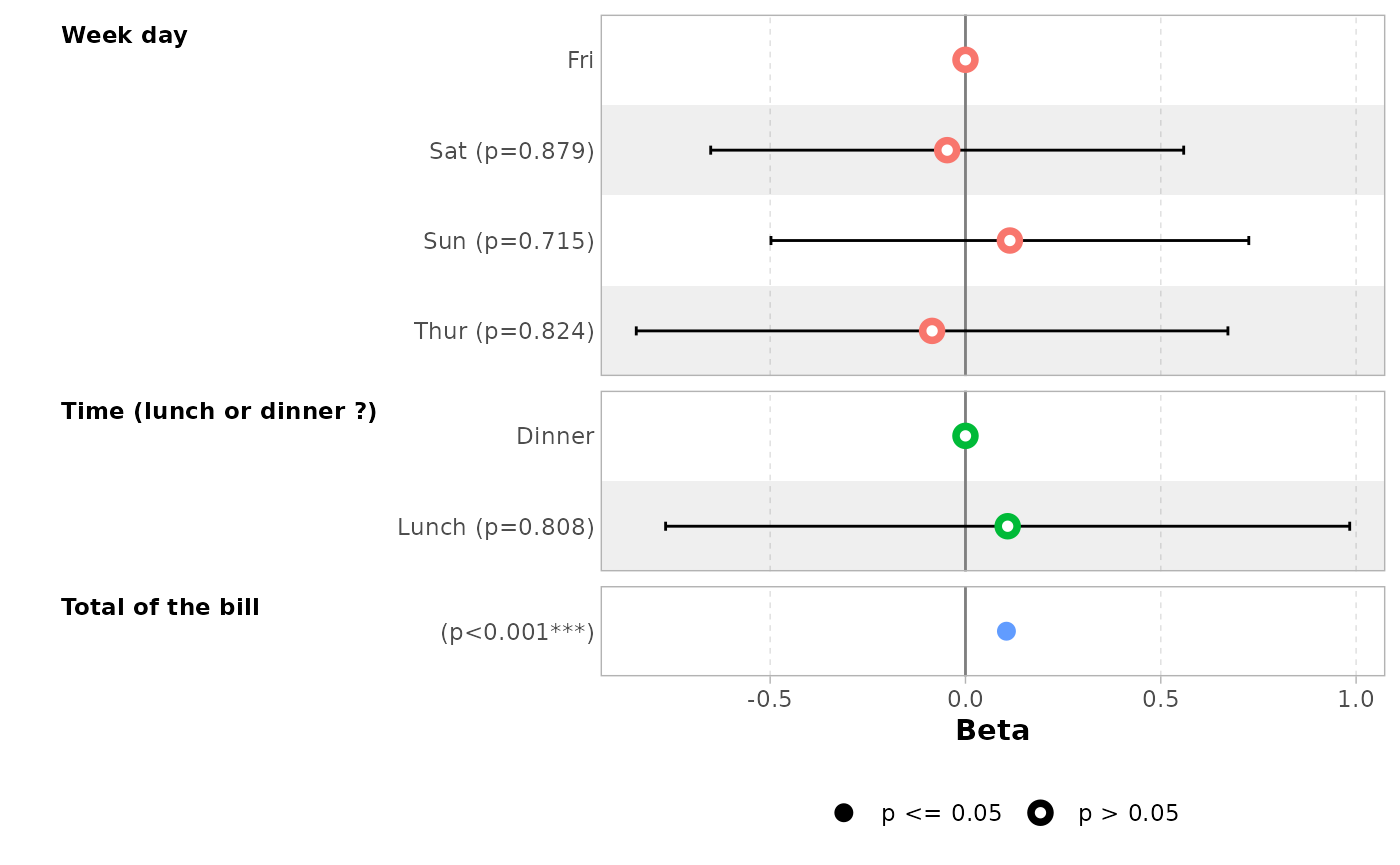Use facet_row = NULL to hide variable names.

ggcoef_model(mod_simple, facet_row = NULL, colour_guide = TRUE)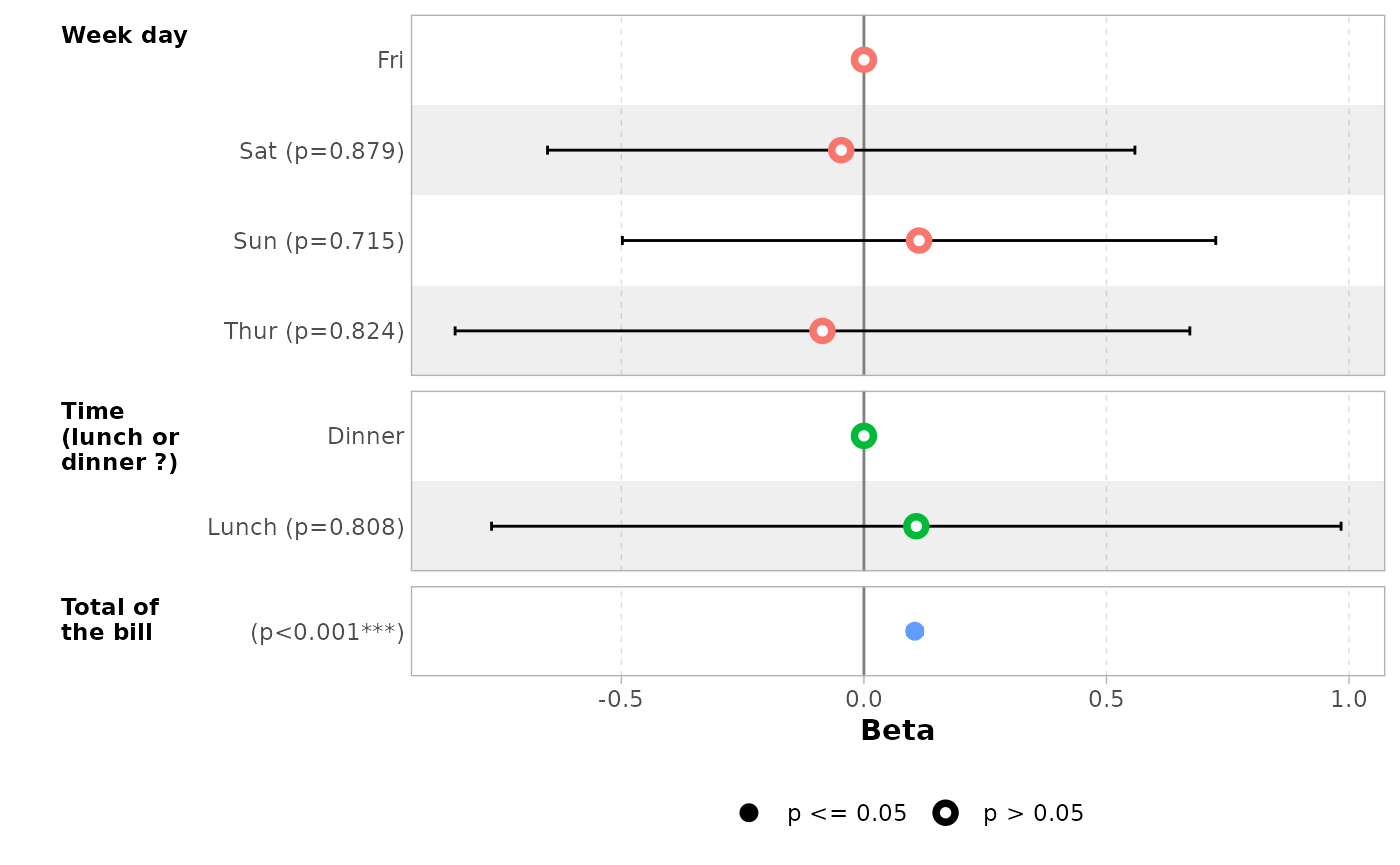### Term labels

Several options allows you to customize term labels.

ggcoef_model(mod_titanic, exponentiate = TRUE)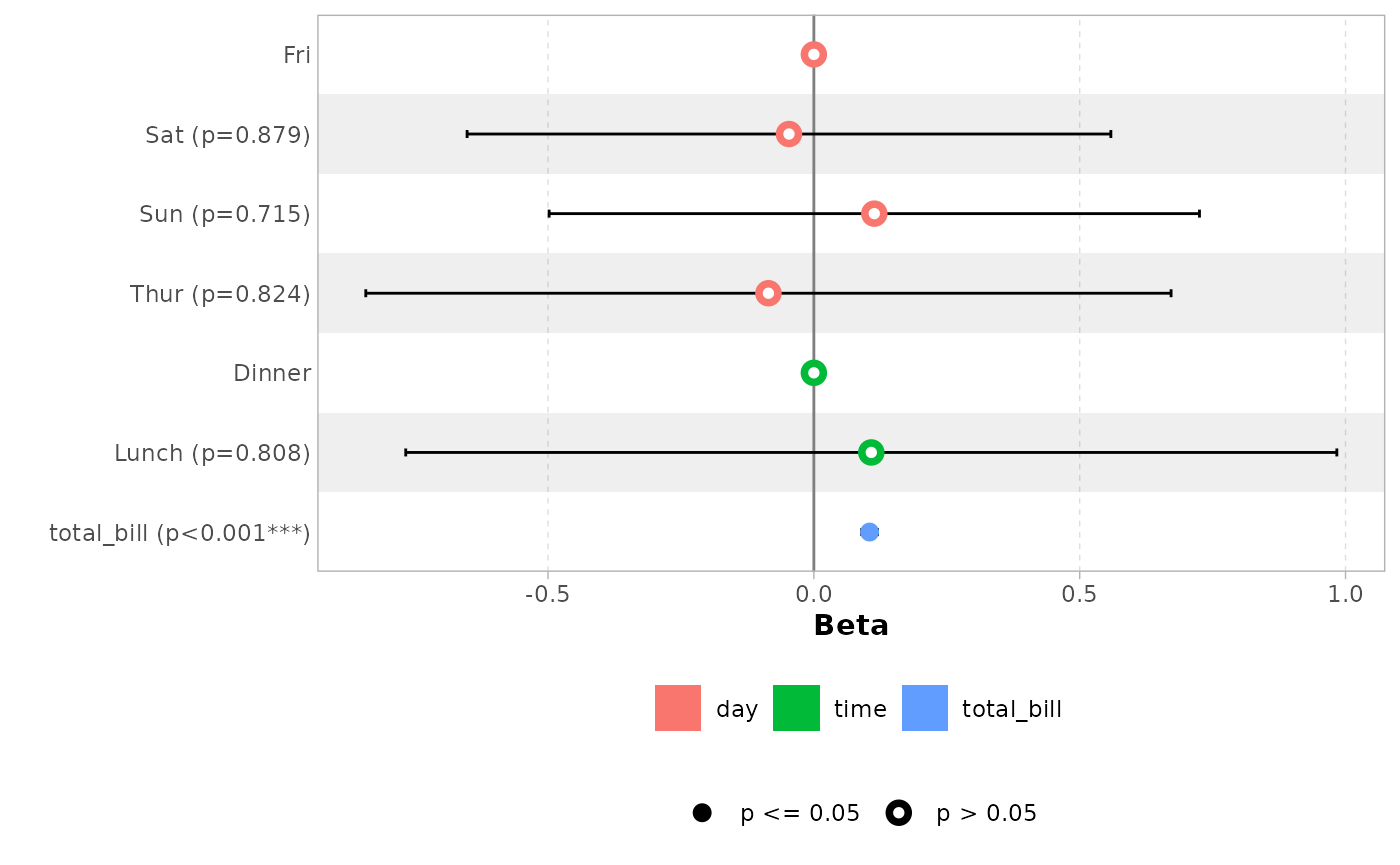ggcoef_model(
mod_titanic,
exponentiate = TRUE,
show_p_values = FALSE,
signif_stars = FALSE,
categorical_terms_pattern = "{level} (ref: {reference_level})",
interaction_sep = " x "
) +
ggplot2::scale_y_discrete(labels = scales::label_wrap(15))
#> Scale for y is already present.
#> Adding another scale for y, which will replace the existing scale.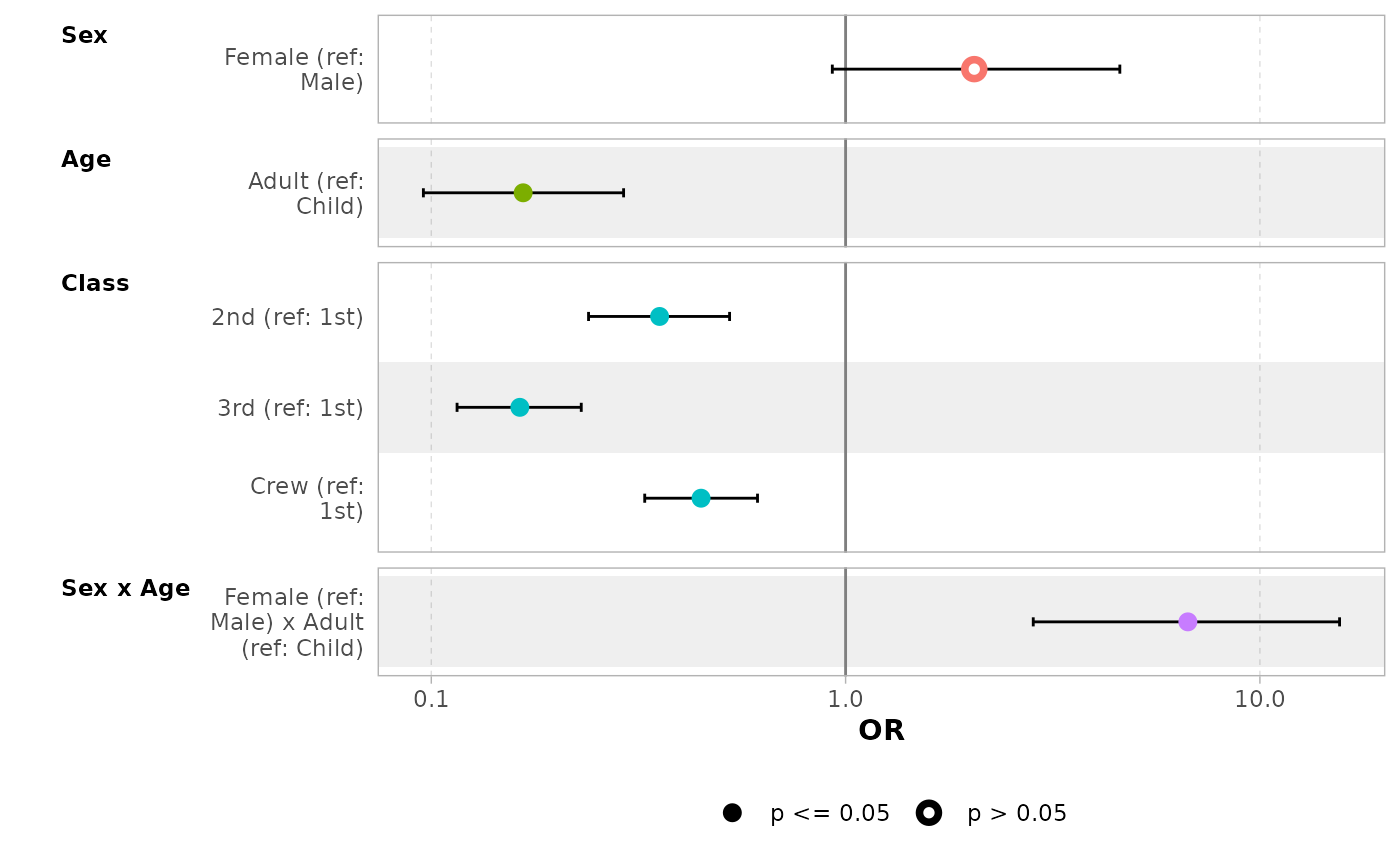By default, for categorical variables using treatment and sum contrasts, reference rows will be added and displayed on the graph.

mod_titanic2 <- glm(
Survived ~ Sex * Age + Class,
weights = Freq,
data = d_titanic,
family = binomial,
contrasts = list(Sex = contr.sum, Class = contr.treatment(4, base = 3))
)
ggcoef_model(mod_titanic2, exponentiate = TRUE)Continuous variables with polynomial terms defined with stats::poly() are also properly managed.

mod_poly <- lm(Sepal.Length ~ poly(Petal.Width, 3) + Petal.Length, data = iris)
ggcoef_model(mod_poly)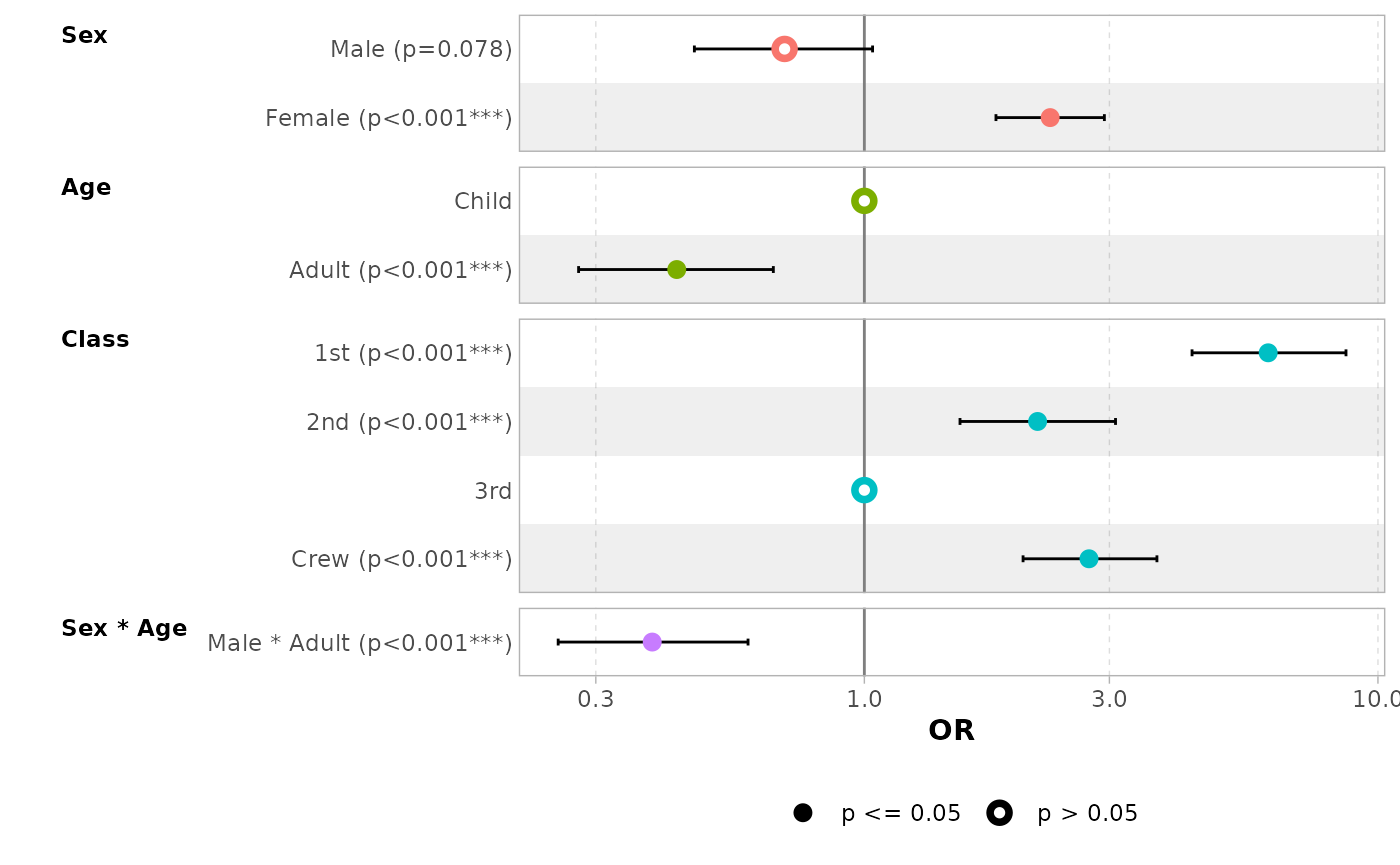Use no_reference_row to indicate which variables should not have a reference row added.

ggcoef_model(
mod_titanic2,
exponentiate = TRUE,
no_reference_row = "Sex"
)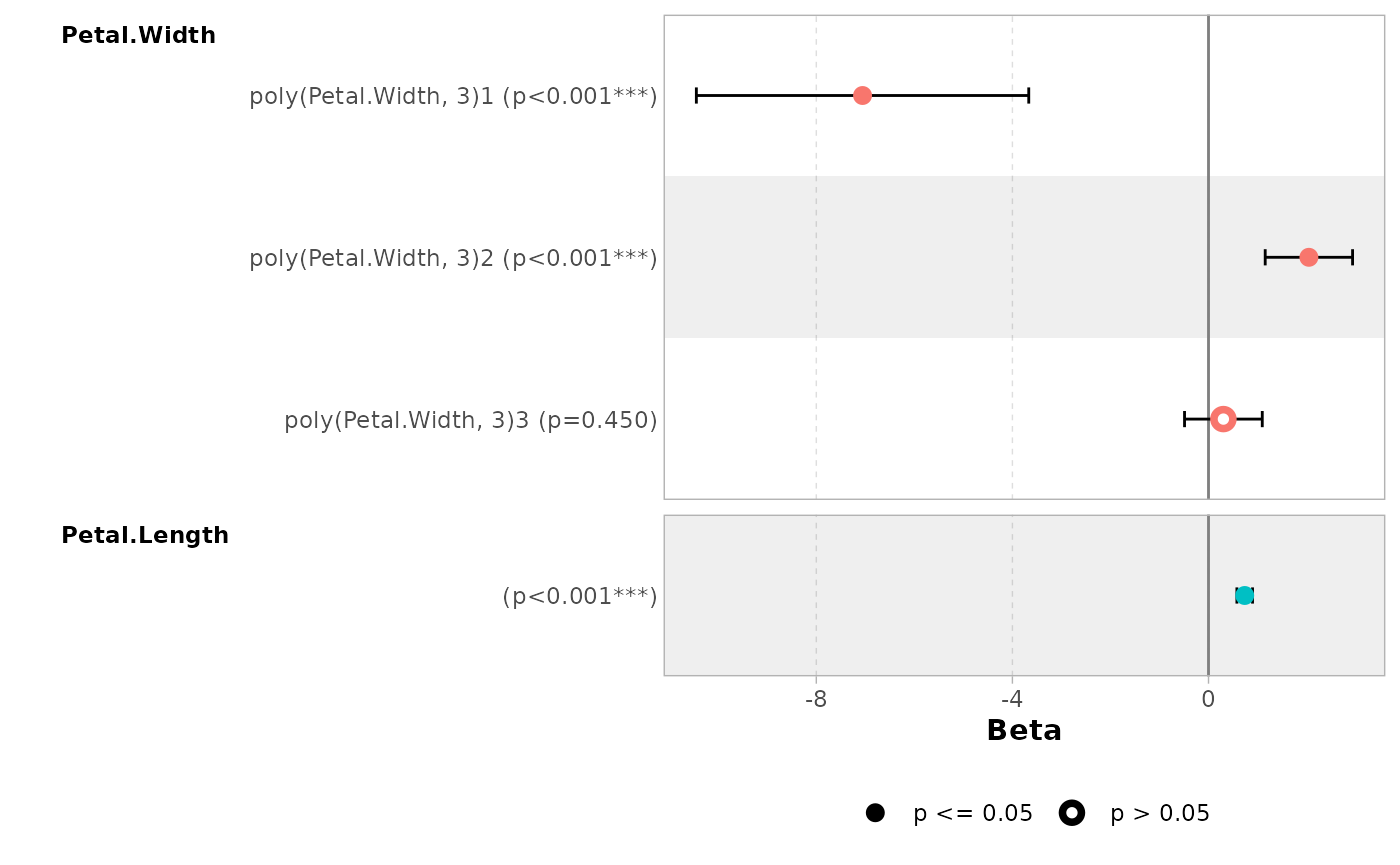ggcoef_model(
mod_titanic2,
exponentiate = TRUE,
no_reference_row = broom.helpers::all_dichotomous()
)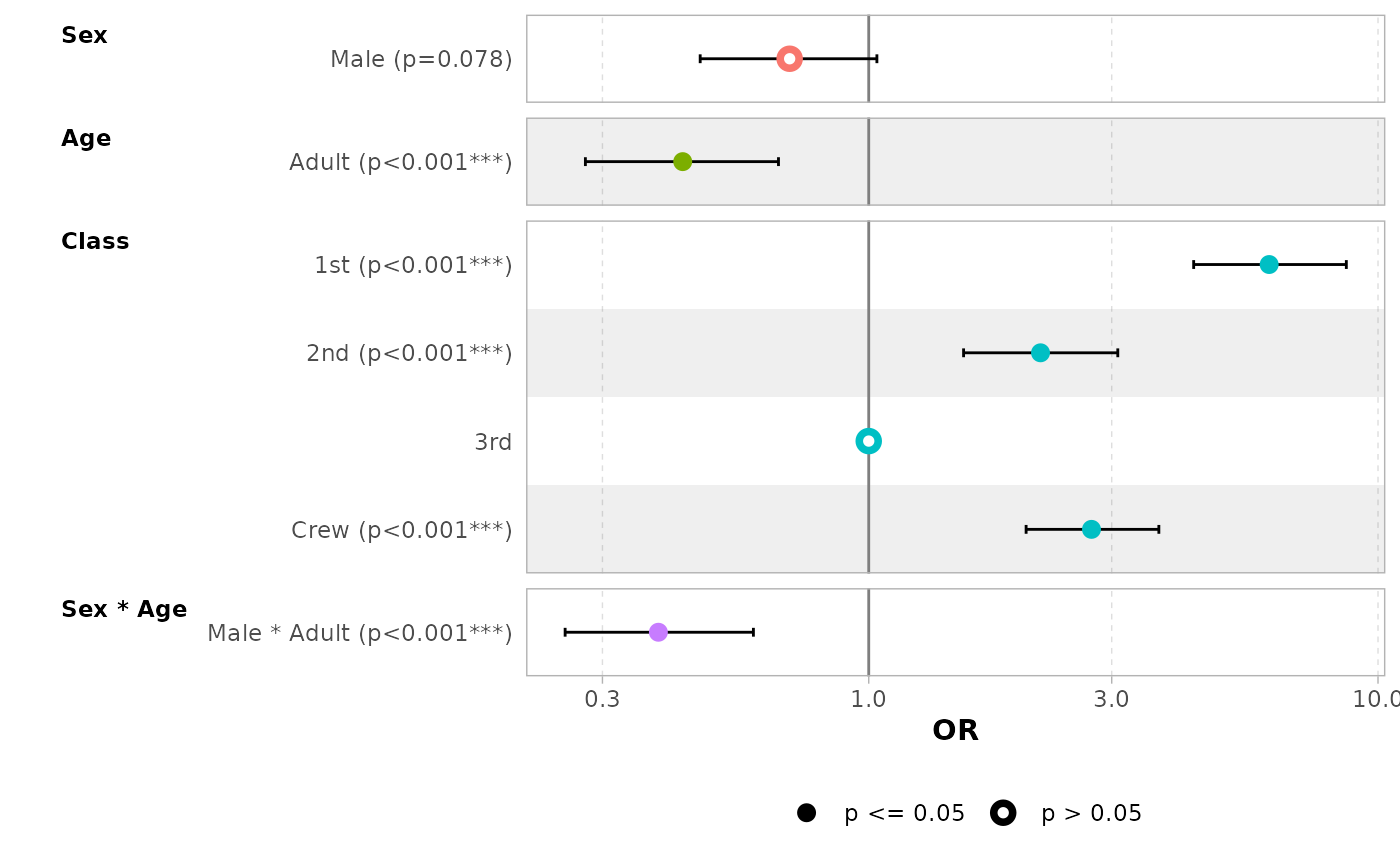ggcoef_model(
mod_titanic2,
exponentiate = TRUE,
no_reference_row = broom.helpers::all_categorical(),
categorical_terms_pattern = "{level}/{reference_level}"
)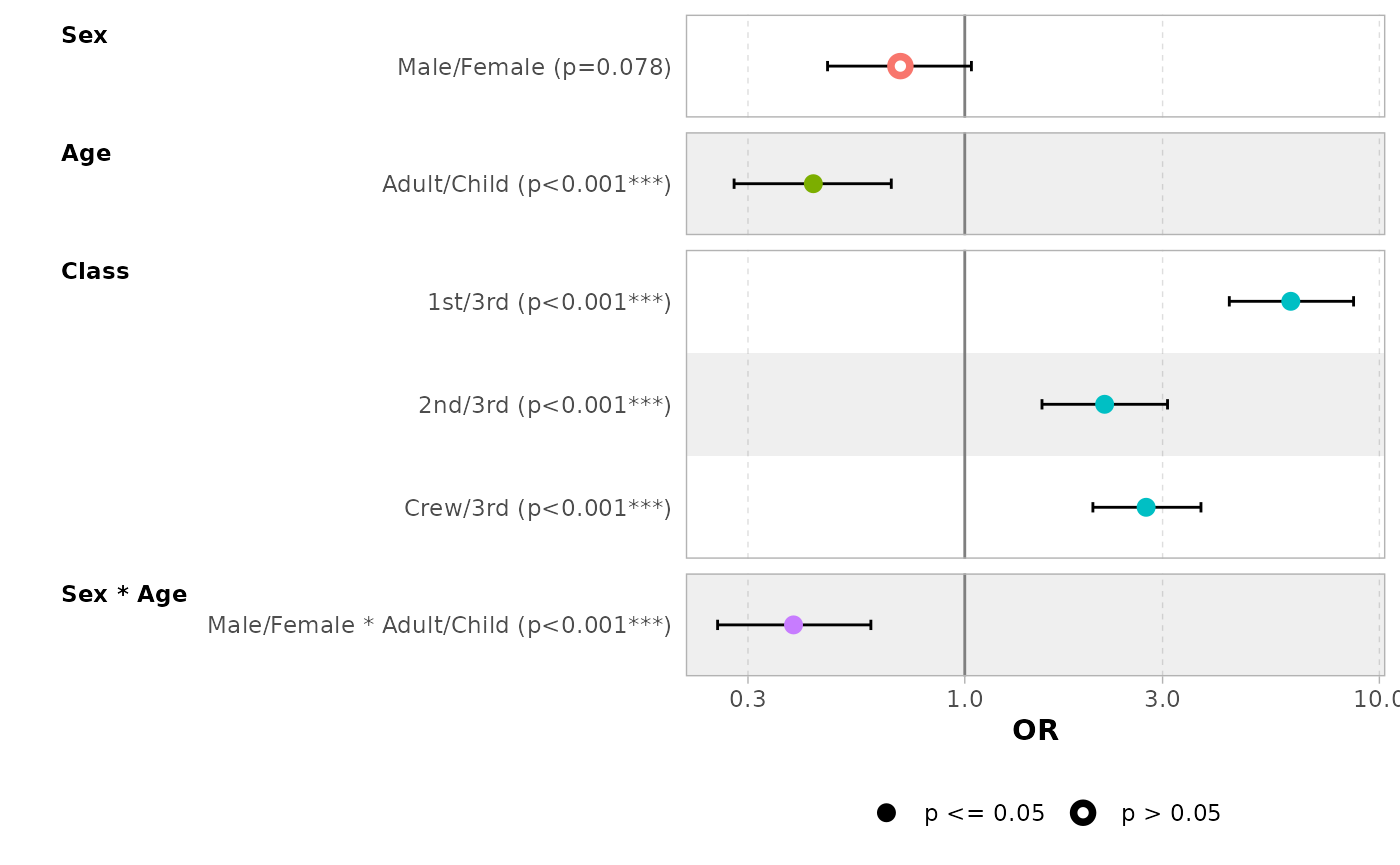### Elements to display

Use intercept = TRUE to display intercepts.

ggcoef_model(mod_simple, intercept = TRUE)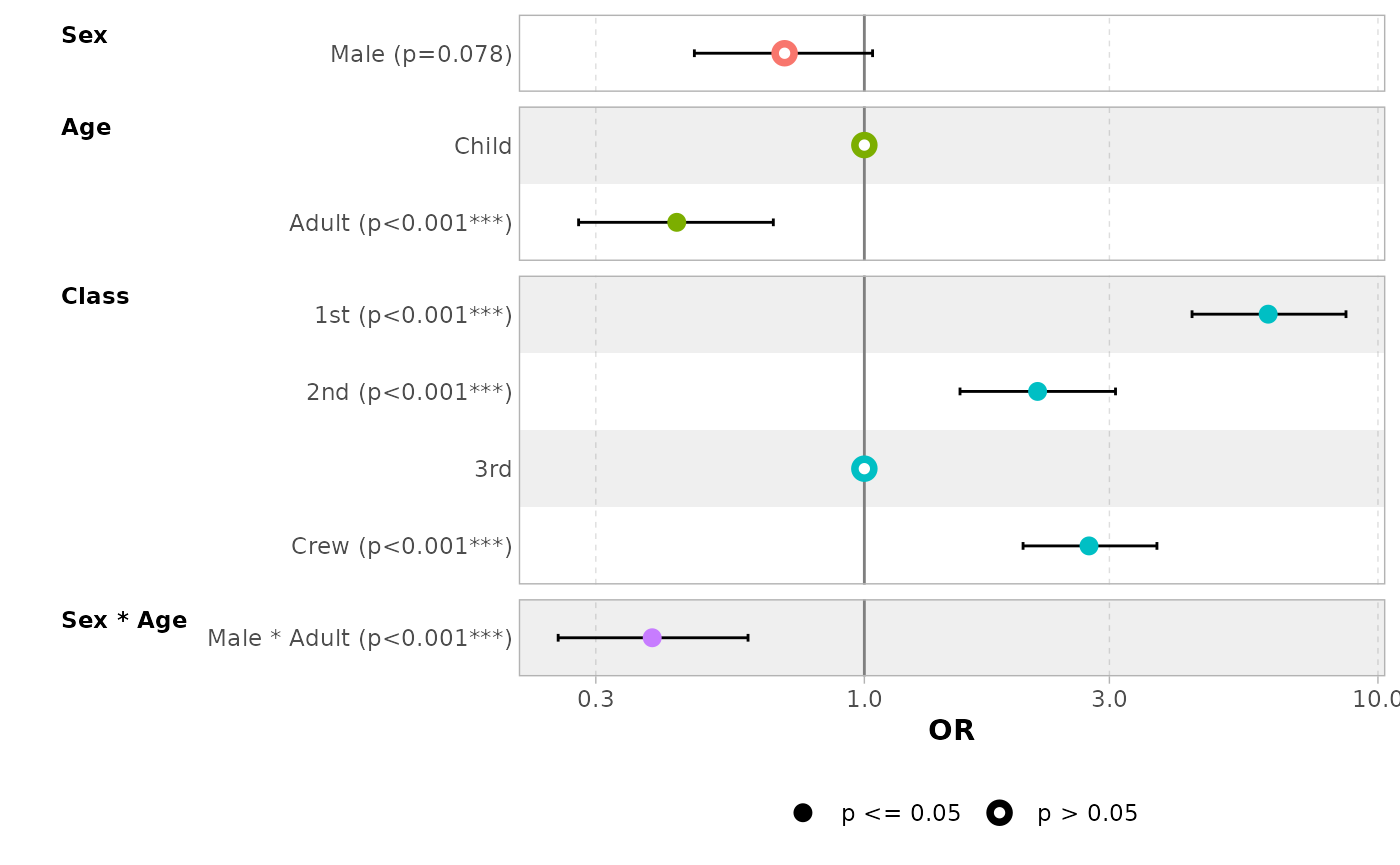You can remove confidence intervals with conf.int = FALSE.

ggcoef_model(mod_simple, conf.int = FALSE)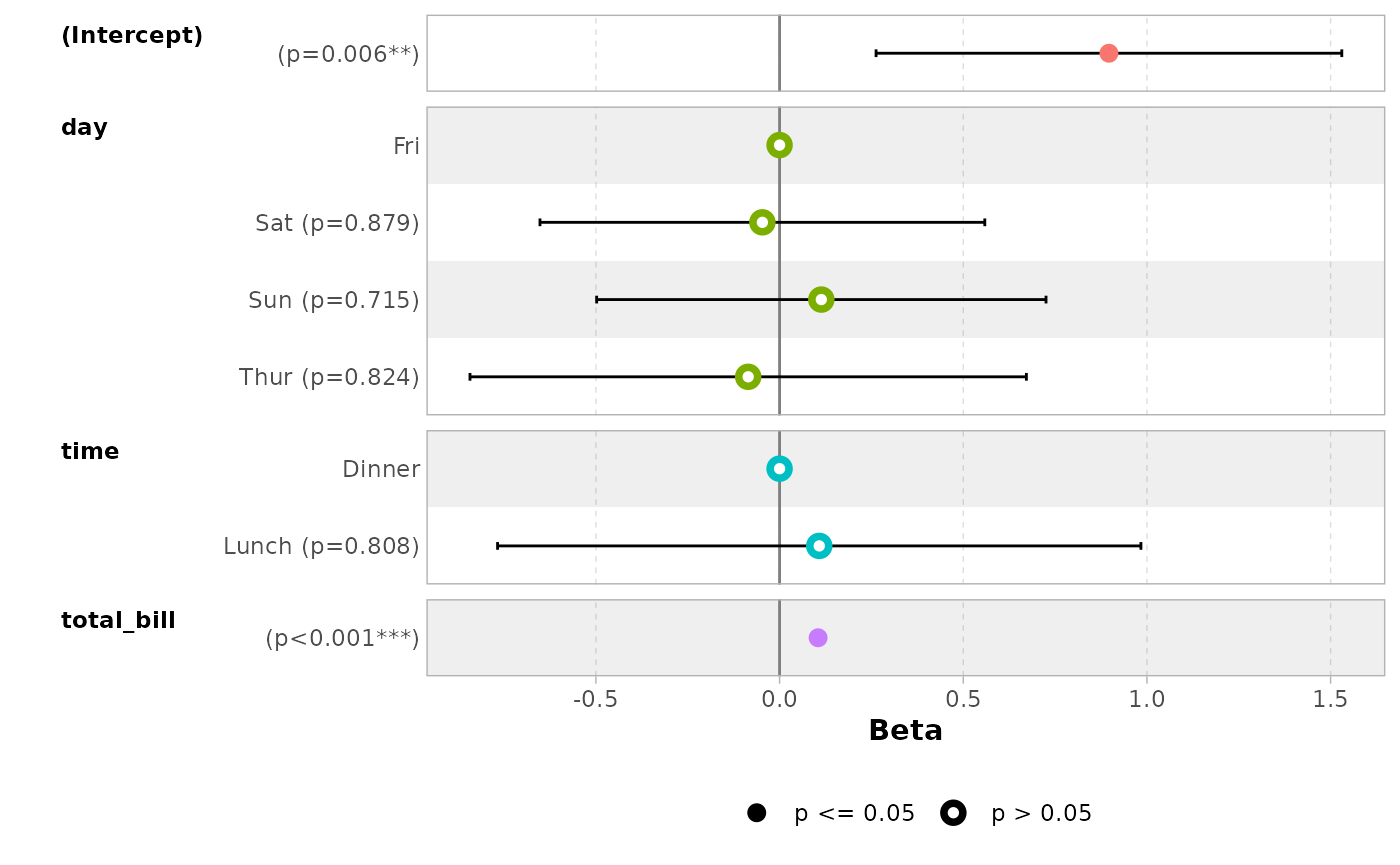By default, significant terms (i.e. with a p-value below 5%) are highlighted using two types of dots. You can control the level of significance with significance or remove it with significance = NULL.

ggcoef_model(mod_simple, significance = NULL)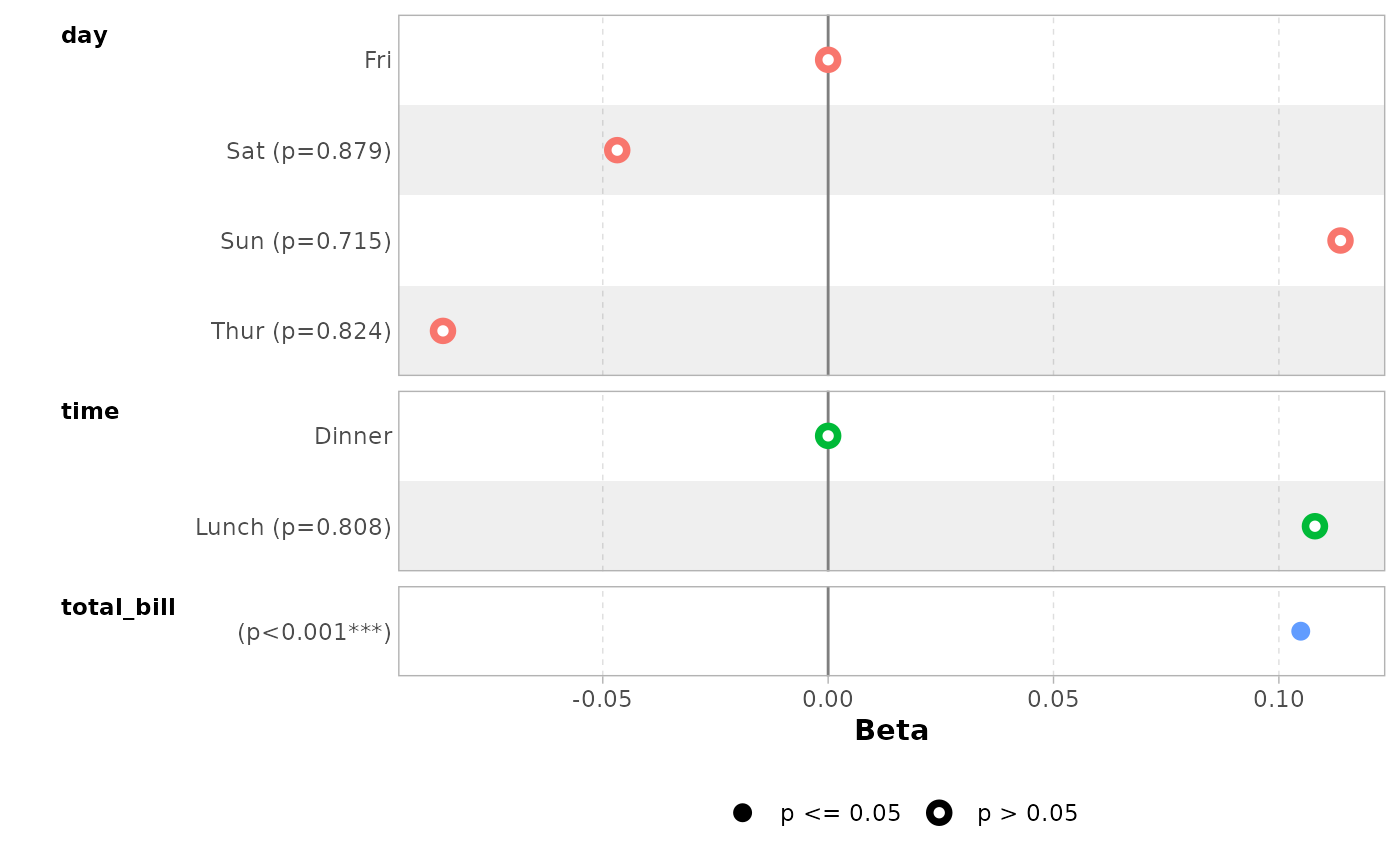By default, dots are colored by variable. You can deactivate this behavior with colour = NULL.

ggcoef_model(mod_simple, colour = NULL)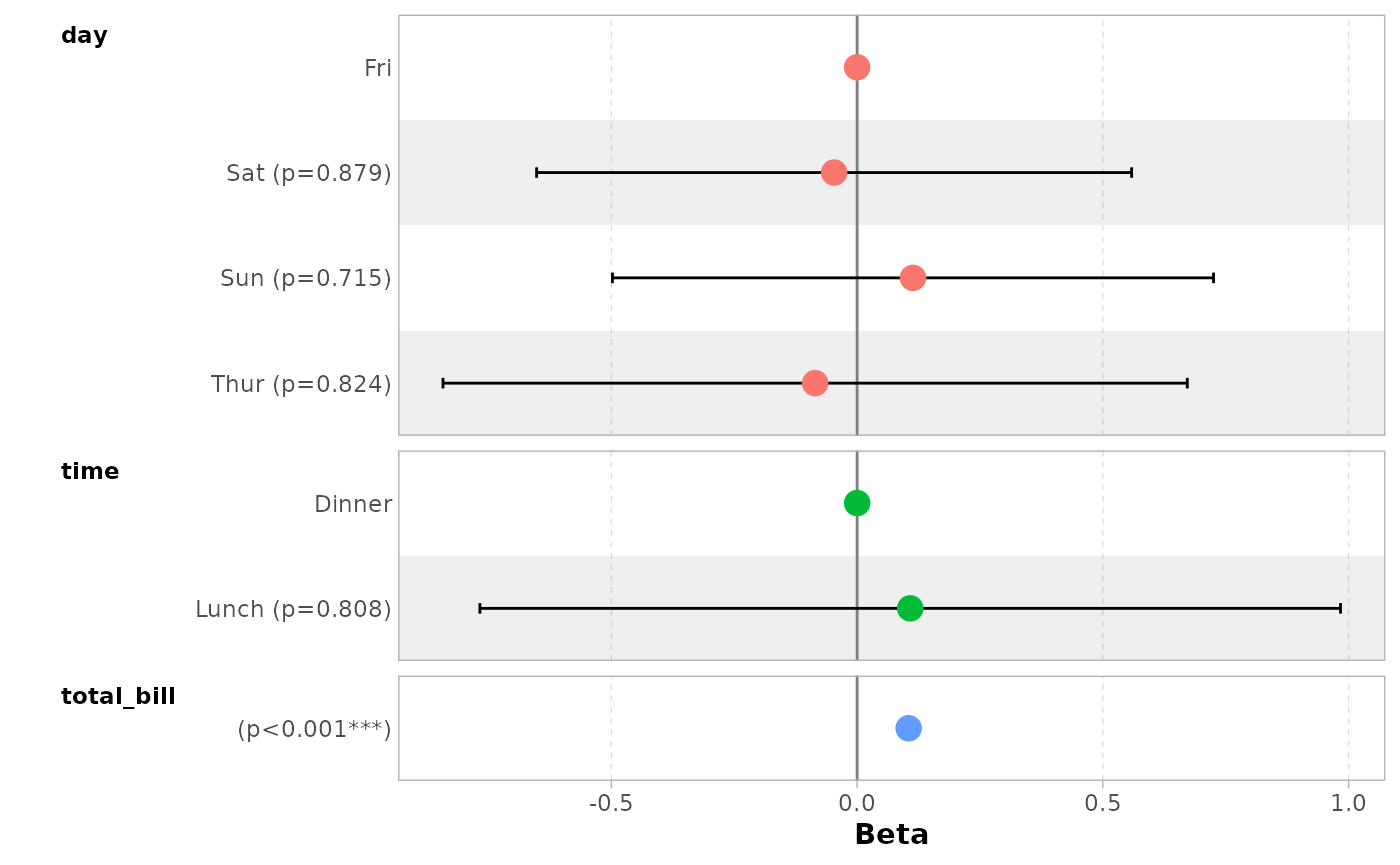You can display only a subset of terms with include.

ggcoef_model(mod_simple, include = c("time", "total_bill"))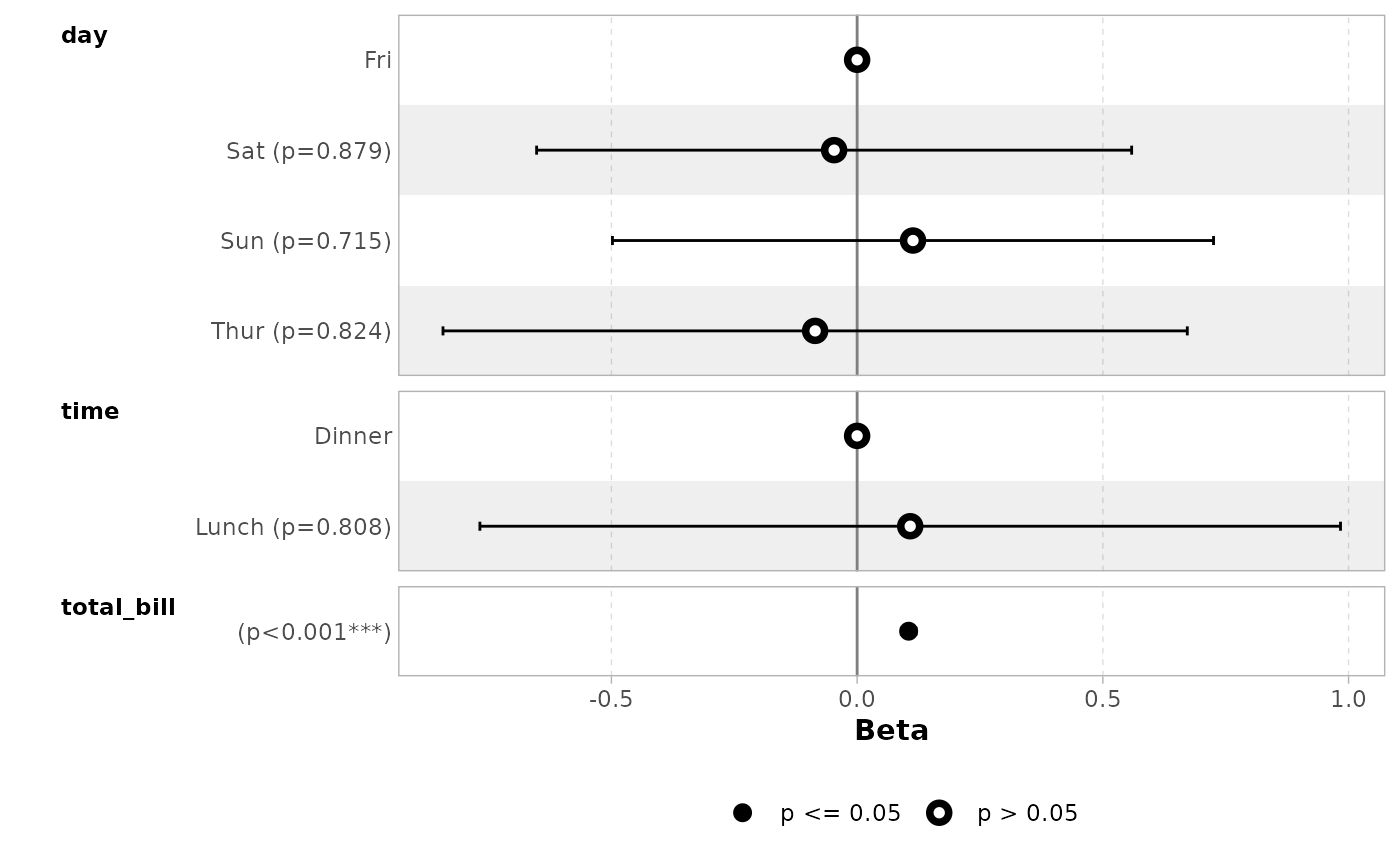It is possible to use tidyselect helpers.

ggcoef_model(mod_simple, include = dplyr::starts_with("t"))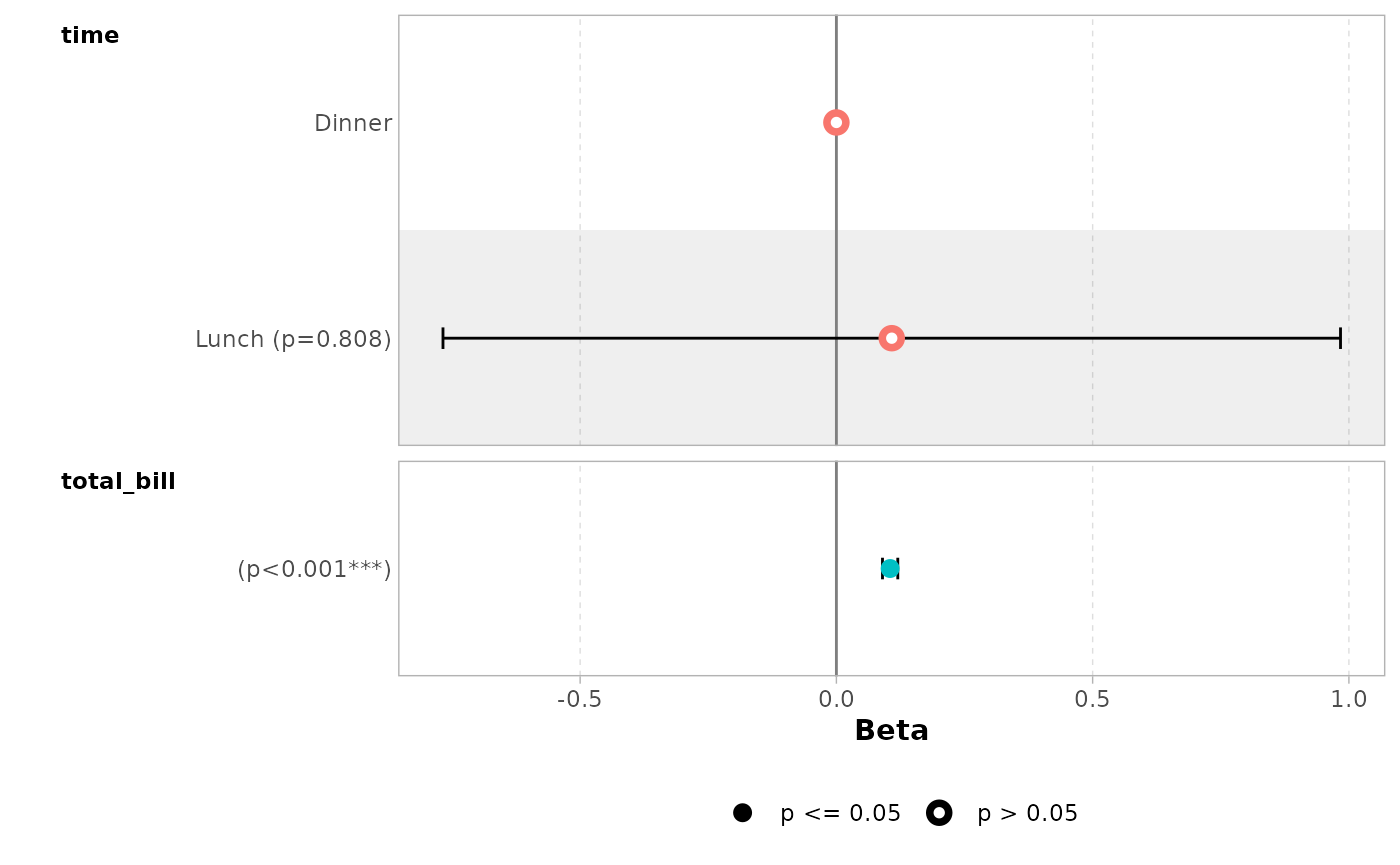You can remove stripped rows with stripped_rows = FALSE.

ggcoef_model(mod_simple, stripped_rows = FALSE)Do not hesitate to consult the help file of ggcoef_model() to see all available options.

### ggplot2 elements

The plot returned by ggcoef_model() is a classic ggplot2 plot. You can therefore apply ggplot2 functions to it.

ggcoef_model(mod_simple) +
ggplot2::xlab("Coefficients") +
ggplot2::ggtitle("Custom title") +
ggplot2::scale_color_brewer(palette = "Set1") +
ggplot2::theme(legend.position = "right")
#> Scale for colour is already present.
#> Adding another scale for colour, which will replace the existing scale.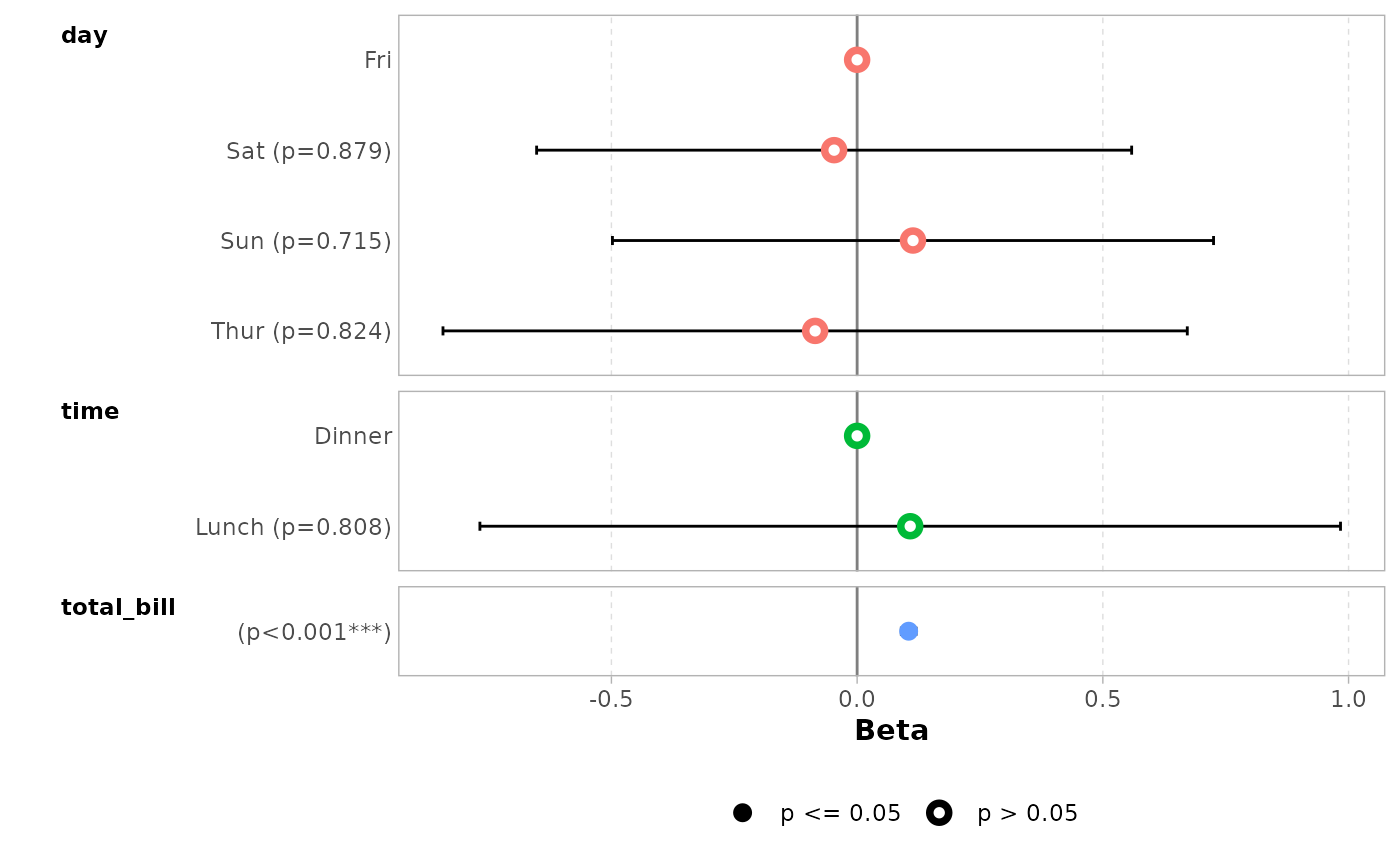## Forest plot with a coefficient table

ggcoef_table() is a variant of ggcoef_model() displaying a coefficient table on the right of the forest plot.

ggcoef_table(mod_simple)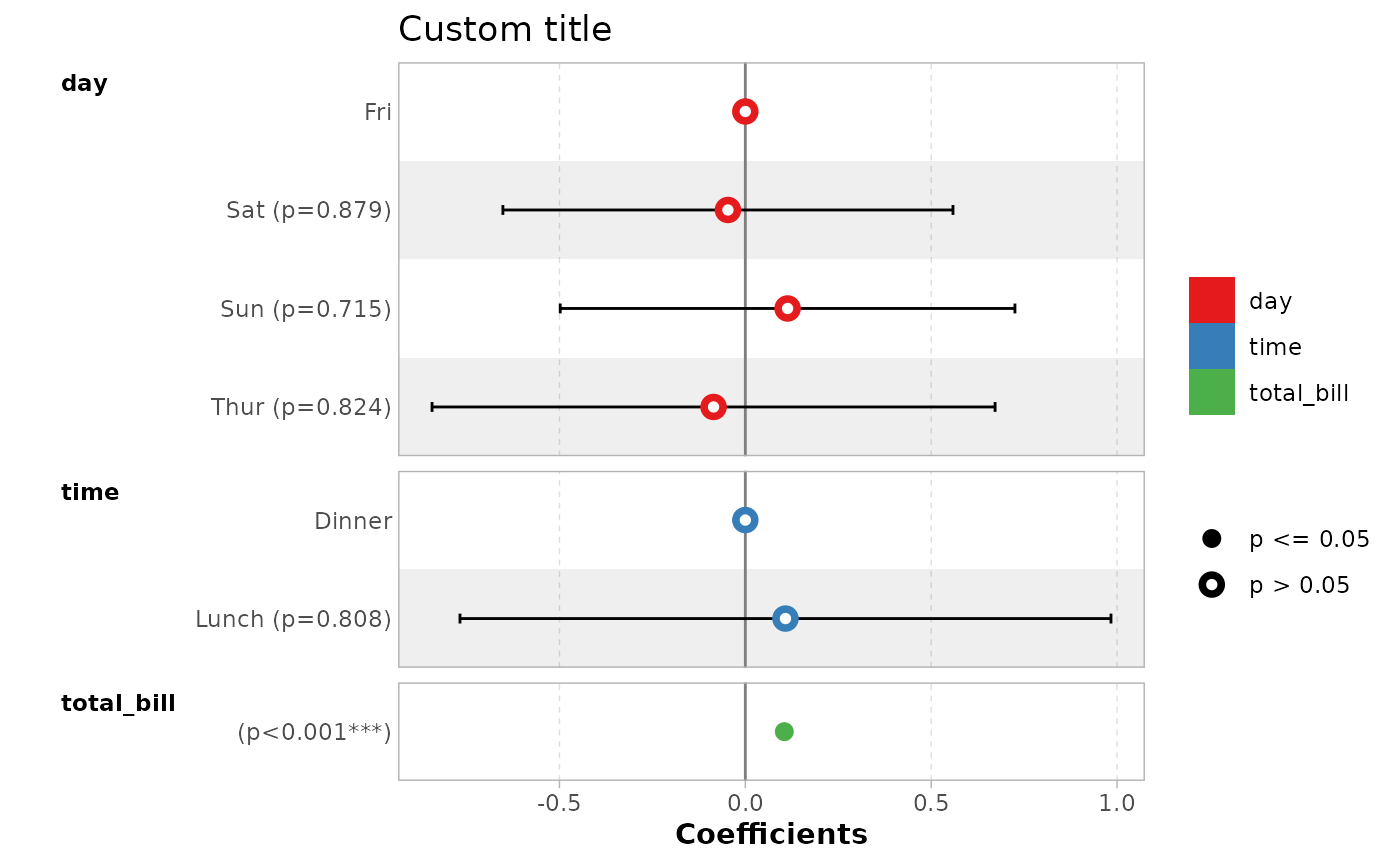ggcoef_table(mod_titanic, exponentiate = TRUE)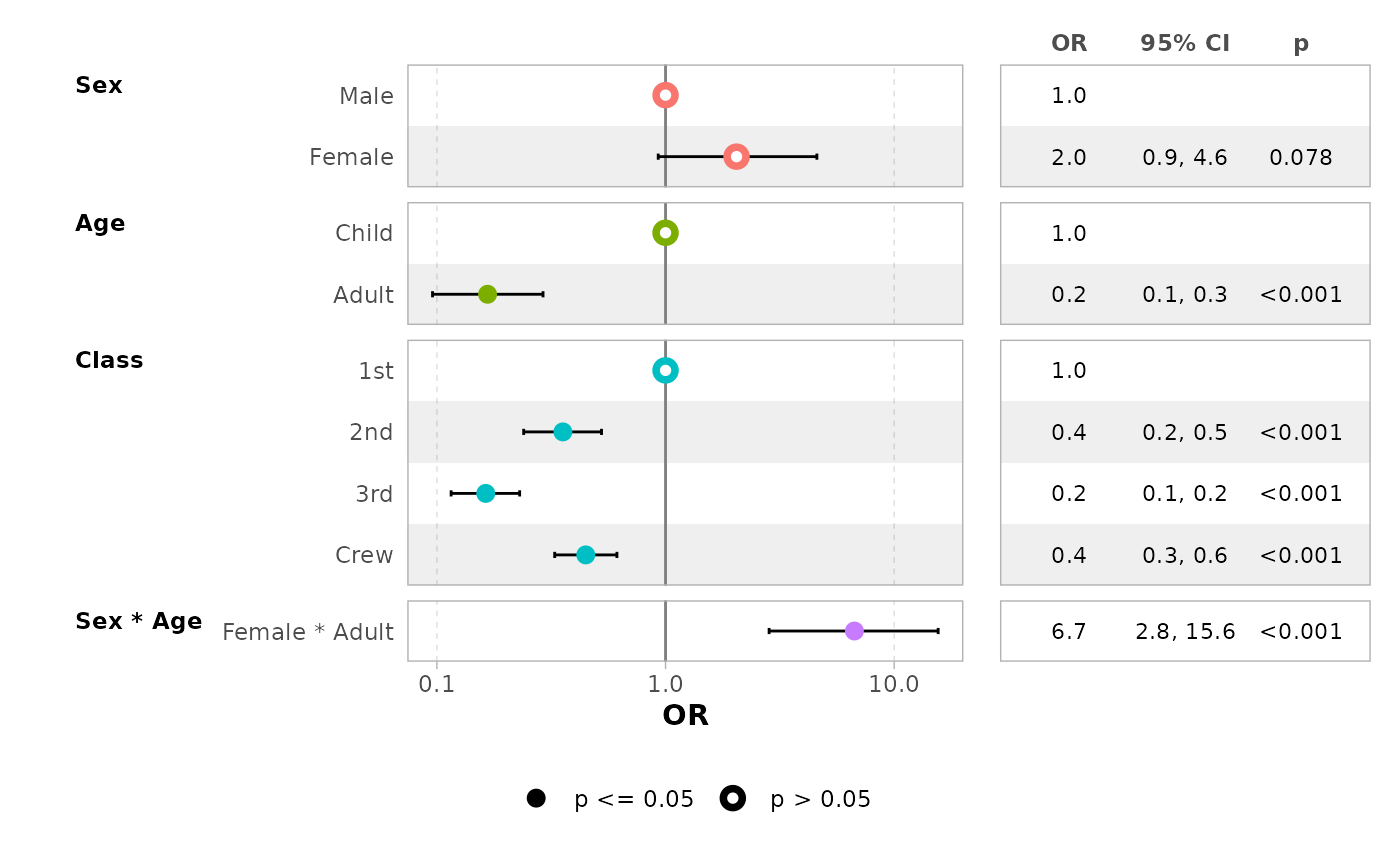You can easily customize the columns to be displayed.

ggcoef_table(
mod_simple,
table_stat = c("label", "estimate", "std.error", "ci"),
ci_pattern = "{conf.low} to {conf.high}",
table_stat_label = list(
estimate = scales::label_number(accuracy = .001),
conf.low = scales::label_number(accuracy = .01),
conf.high = scales::label_number(accuracy = .01),
std.error = scales::label_number(accuracy = .001),
label = toupper
),
table_header = c("Term", "Coef.", "SE", "CI"),
table_witdhs = c(2, 3)
)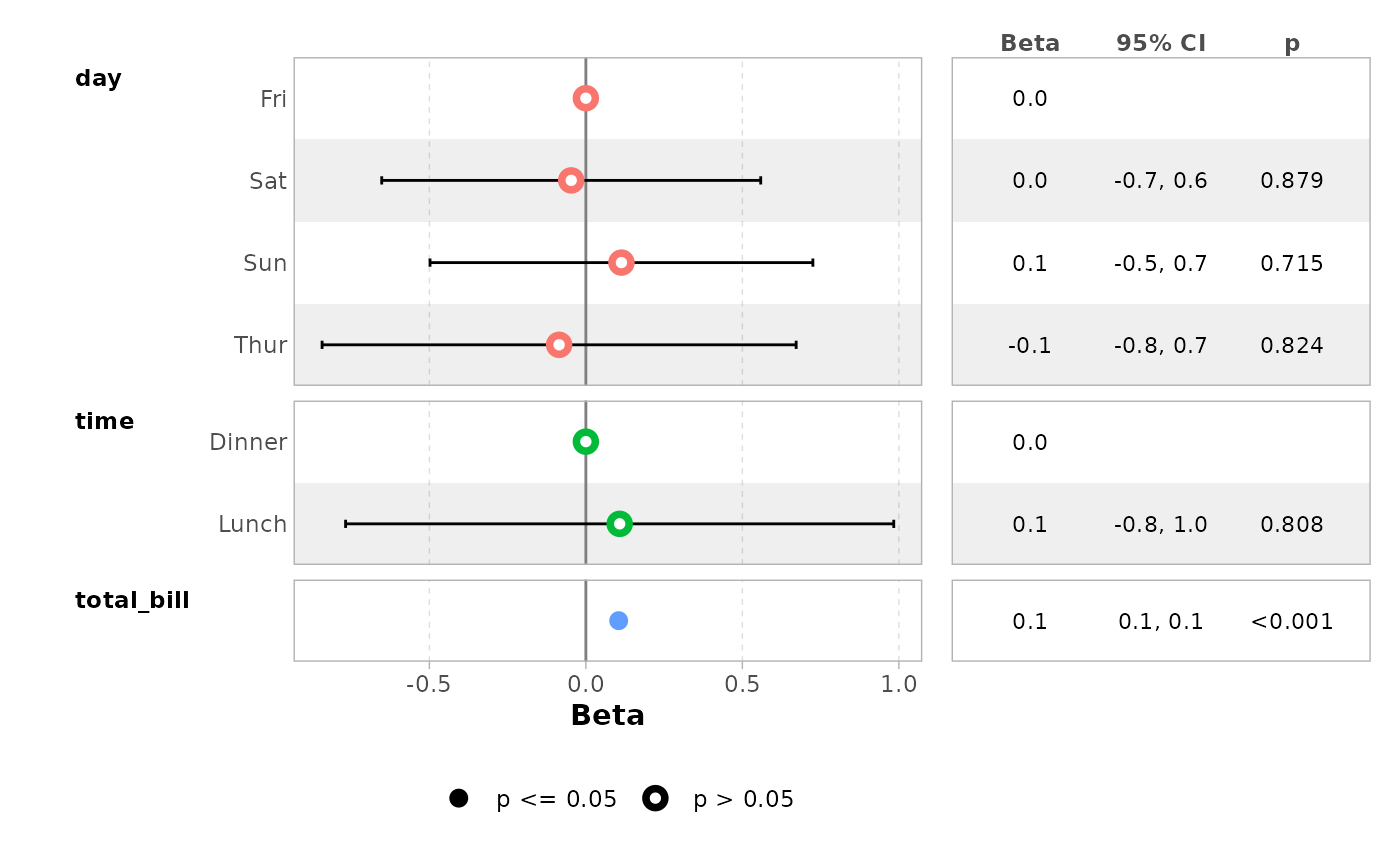## Multinomial models

For multinomial models, simply use ggcoef_multinom(). Three types of visualizations are available: "dodged", "faceted" and "table".

library(nnet)
hec <- as.data.frame(HairEyeColor)
mod <- multinom(
Hair ~ Eye + Sex,
data = hec,
weights = hec\$Freq
)
#> # weights:  24 (15 variable)
#> initial  value 820.686262
#> iter  10 value 669.061500
#> iter  20 value 658.888977
#> final  value 658.885327
#> converged
ggcoef_multinom(
mod,
exponentiate = TRUE
)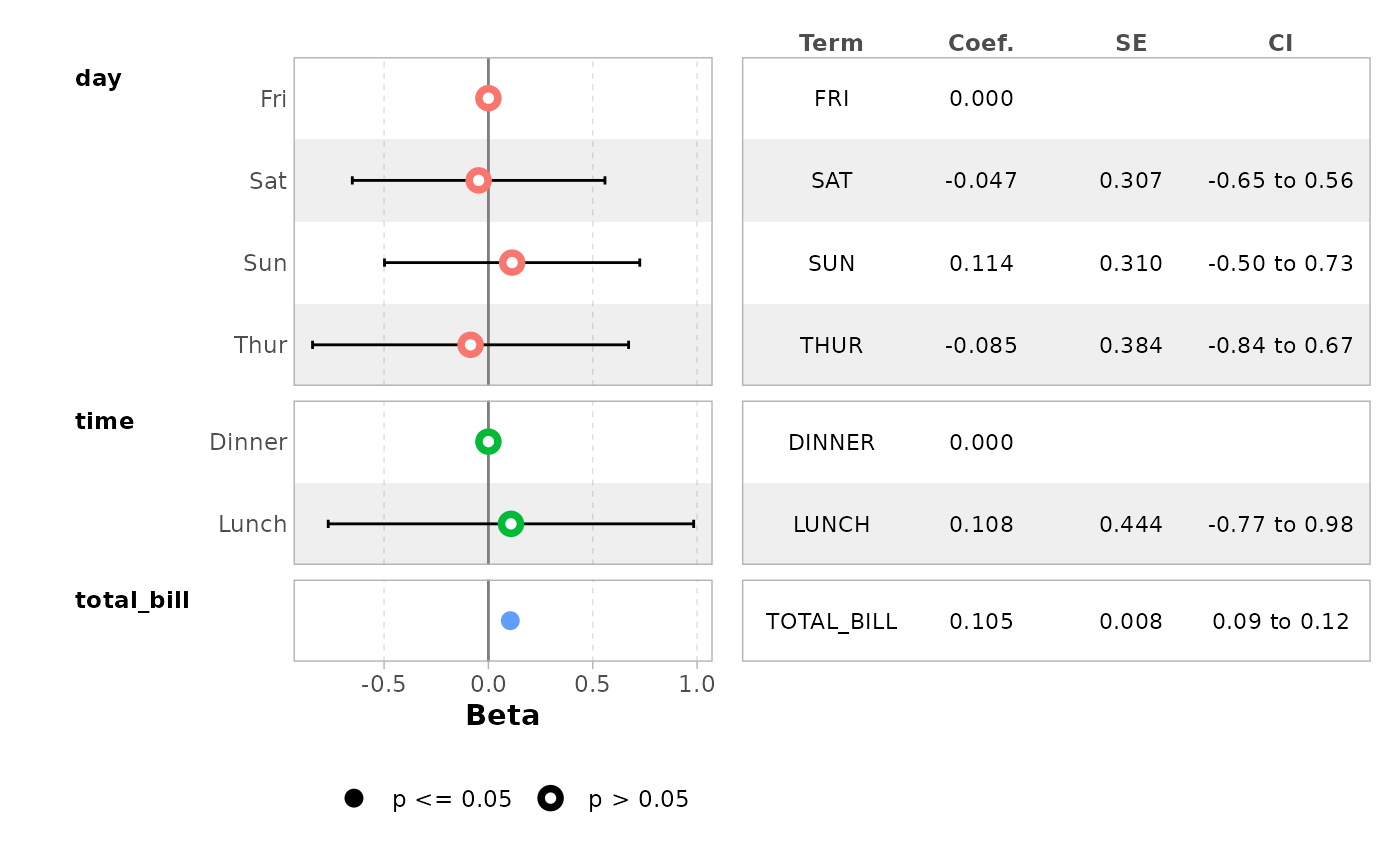ggcoef_multinom(
mod,
exponentiate = TRUE,
type = "faceted"
)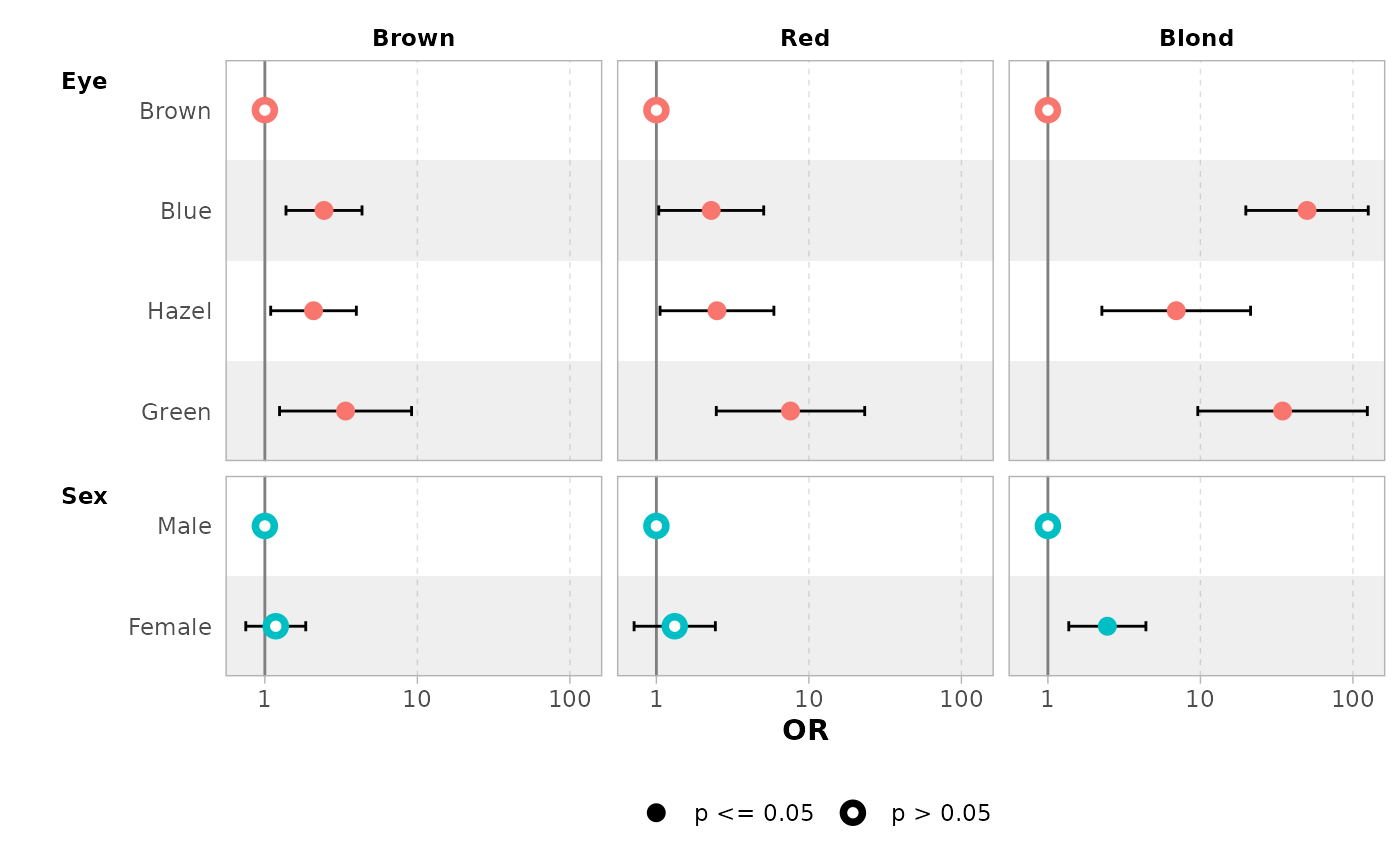ggcoef_multinom(
mod,
exponentiate = TRUE,
type = "table"
)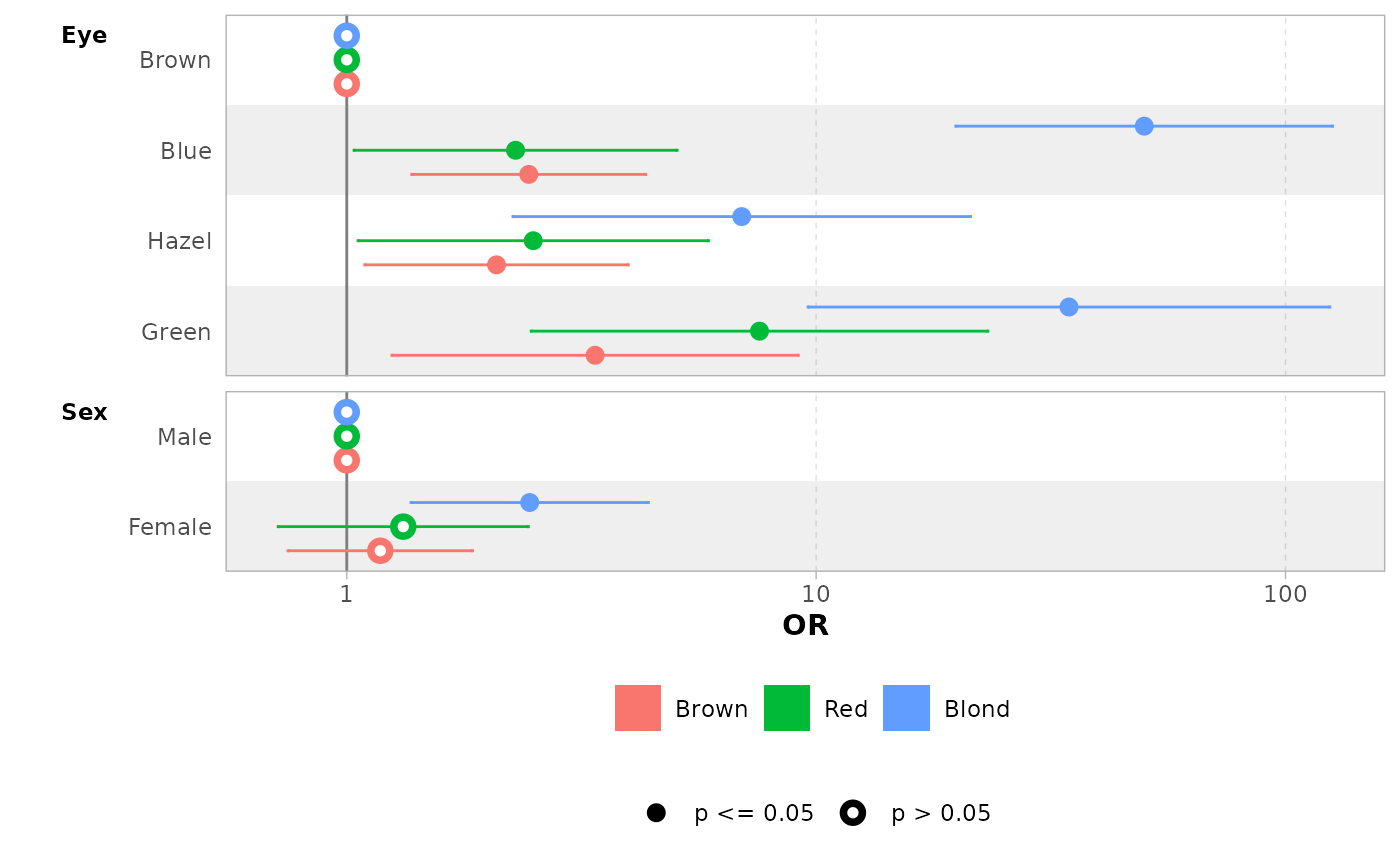You can use y.level_label to customize the label of each level.

ggcoef_multinom(
mod,
type = "faceted",
y.level_label = c("Brown" = "Brown\n(ref: Black)"),
exponentiate = TRUE
)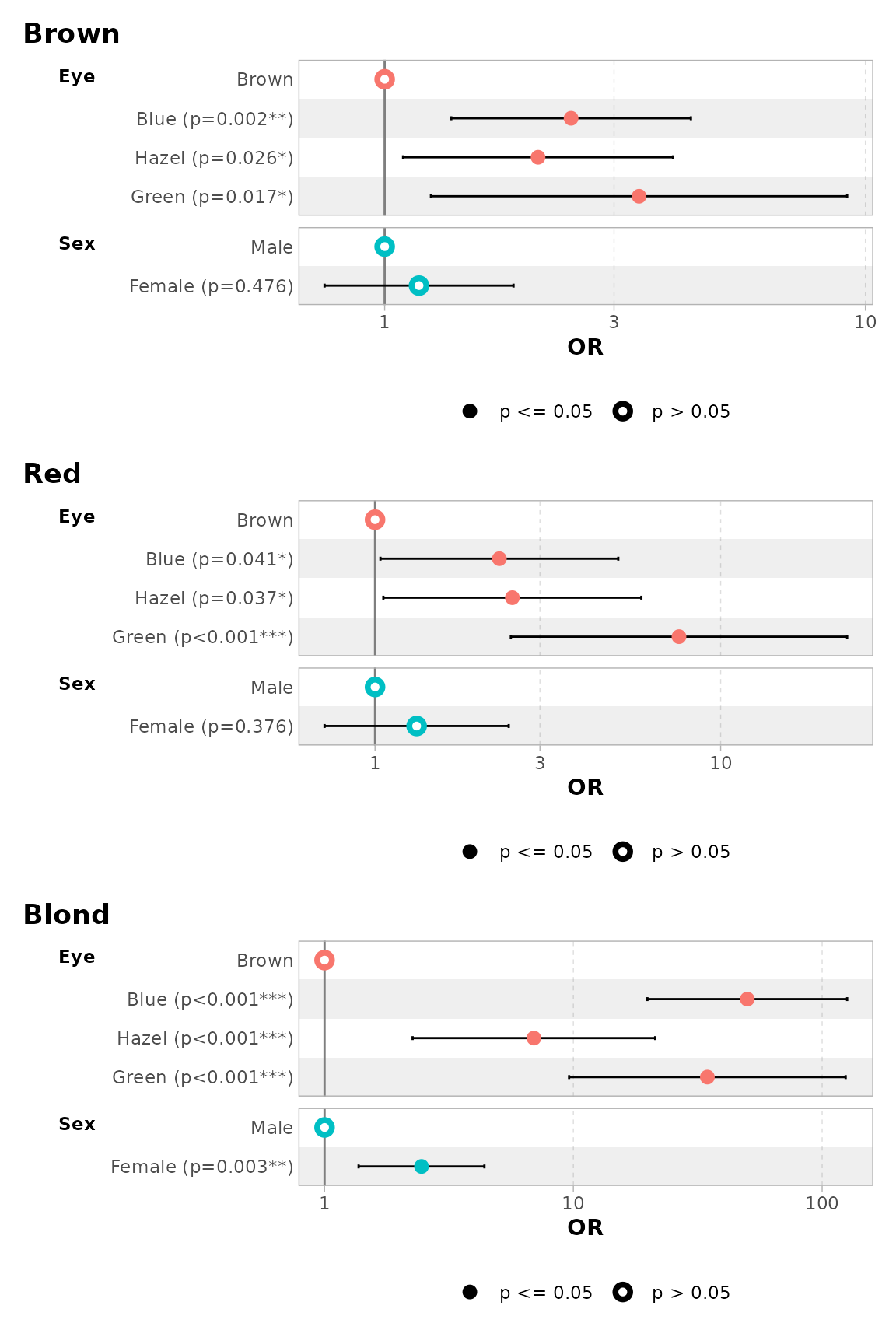## Multi-components models

Multi-components models such as zero-inflated Poisson or beta regression generate a set of terms for each of their components. You can use ggcoef_multicomponents() which is similar to ggcoef_multinom().

library(pscl)
#> Classes and Methods for R developed in the
#> Political Science Computational Laboratory
#> Department of Political Science
#> Stanford University
#> Simon Jackman
#> hurdle and zeroinfl functions by Achim Zeileis
data("bioChemists", package = "pscl")
mod <- zeroinfl(art ~ fem * mar | fem + mar, data = bioChemists)

ggcoef_multicomponents(mod)
#> ℹ <zeroinfl> model detected.
#> ✔ tidy_zeroinfl() used instead.
#> ℹ Add tidy_fun = broom.helpers::tidy_zeroinfl to quiet these messages.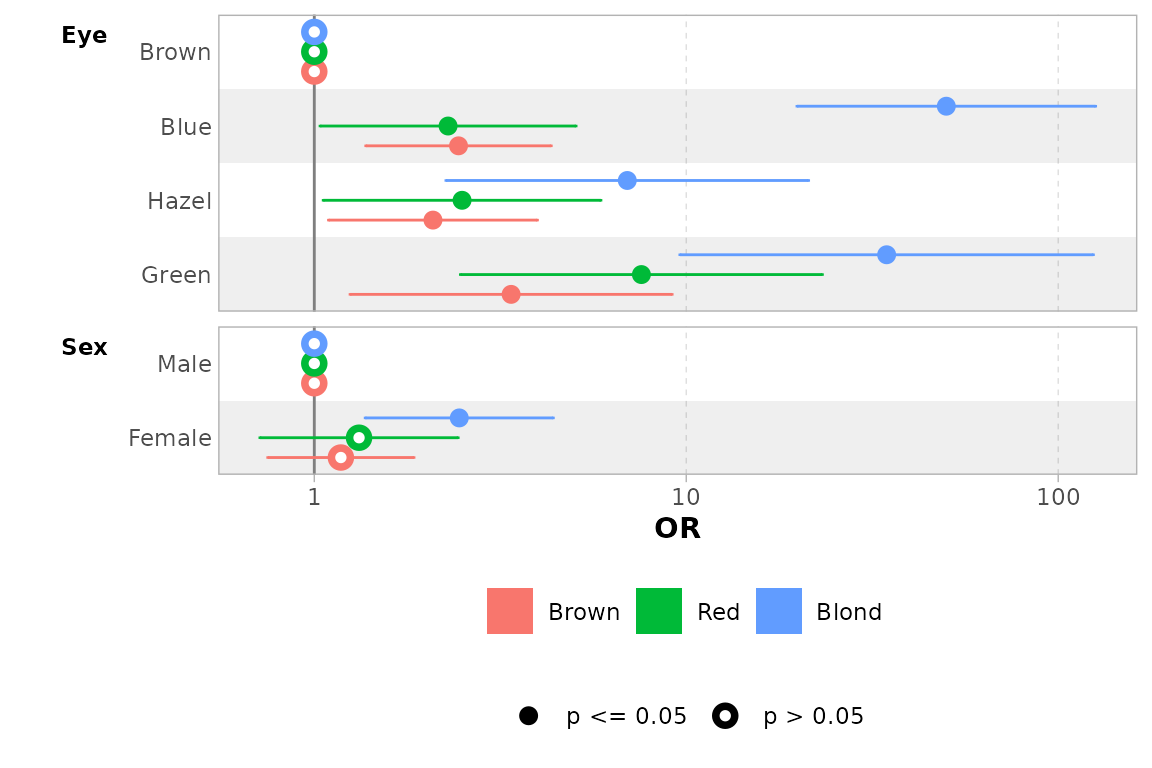ggcoef_multicomponents(mod, type = "f")
#> ℹ <zeroinfl> model detected.
#> ✔ tidy_zeroinfl() used instead.
#> ℹ Add tidy_fun = broom.helpers::tidy_zeroinfl to quiet these messages.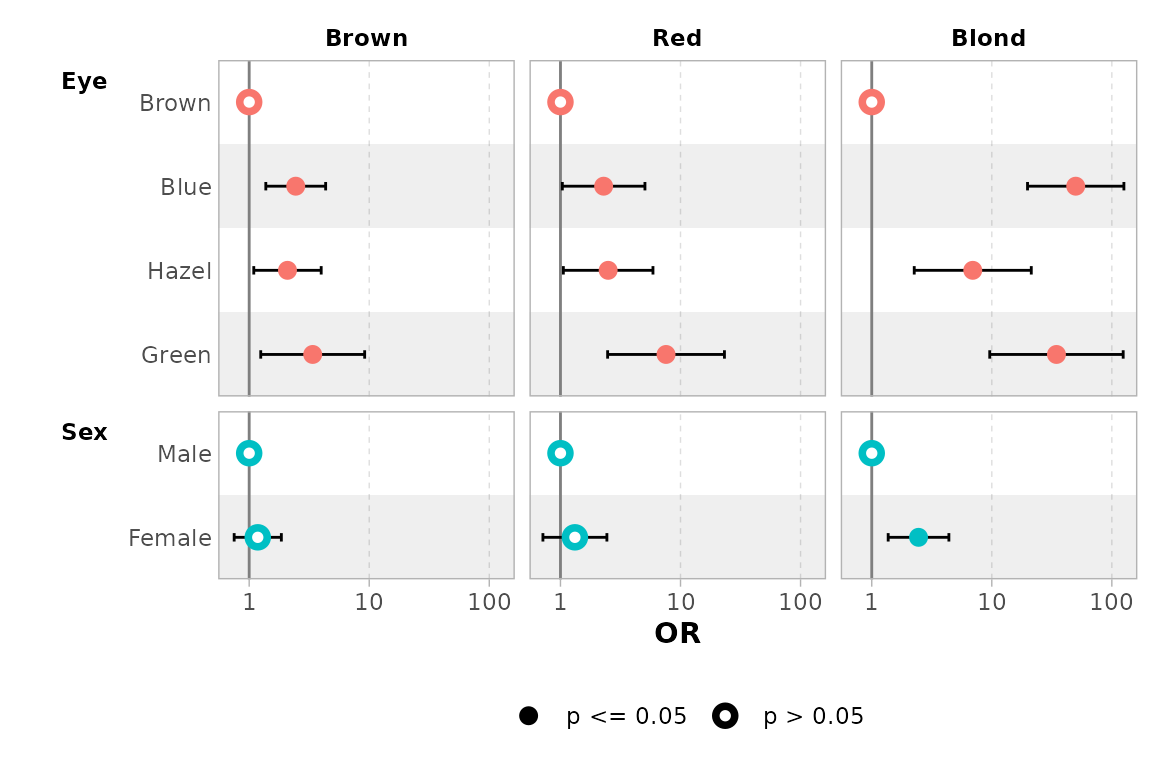ggcoef_multicomponents(mod, type = "t")
#> ℹ <zeroinfl> model detected.
#> ✔ tidy_zeroinfl() used instead.
#> ℹ Add tidy_fun = broom.helpers::tidy_zeroinfl to quiet these messages.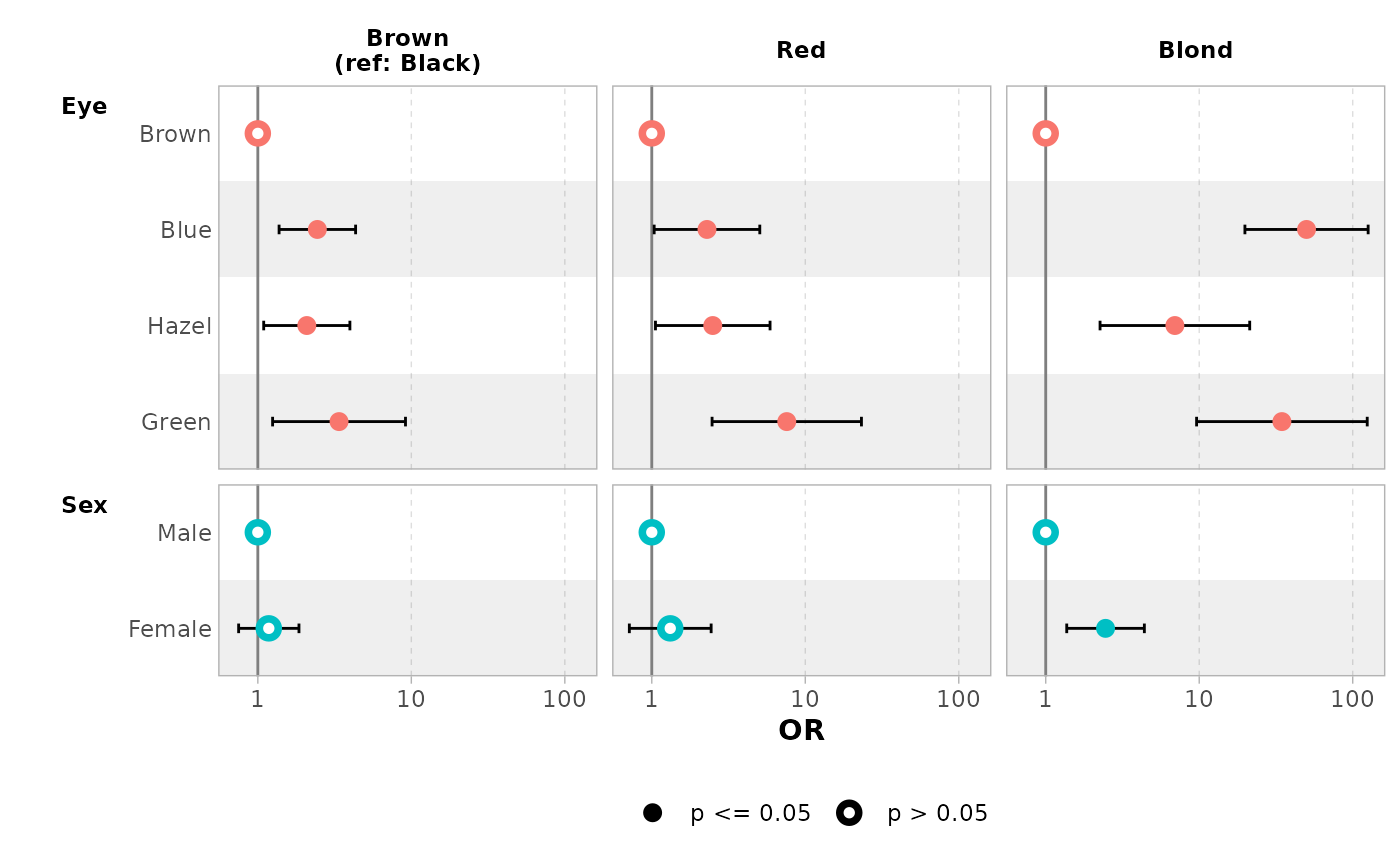ggcoef_multicomponents(
mod,
type = "t",
component_label = c(conditional = "Count", zero_inflated = "Zero-inflated")
)
#> ℹ <zeroinfl> model detected.
#> ✔ tidy_zeroinfl() used instead.
#> ℹ Add tidy_fun = broom.helpers::tidy_zeroinfl to quiet these messages.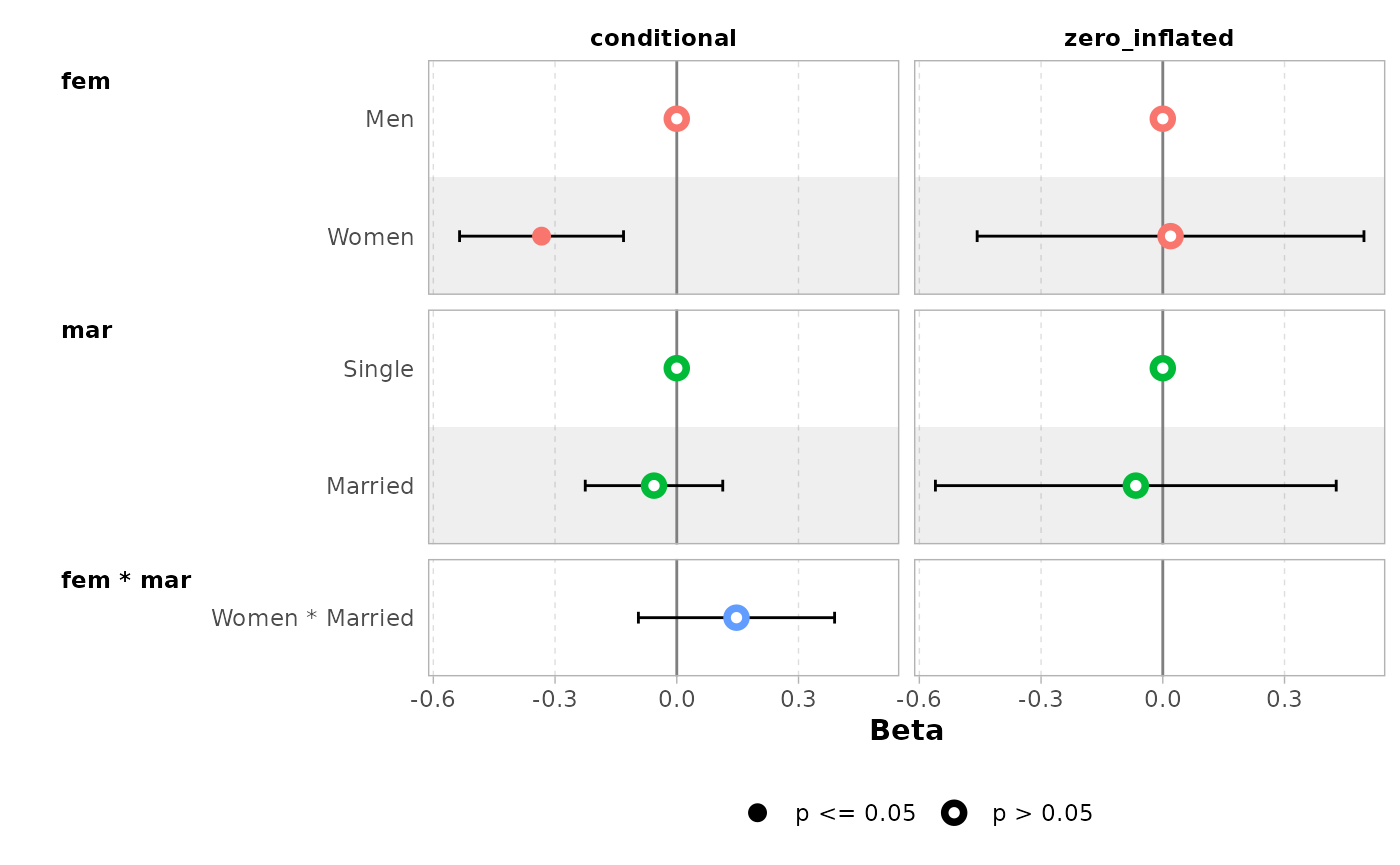## Comparing several models

You can easily compare several models with ggcoef_compare(). To be noted, ggcoef_compare() is not compatible with multinomial or multi-components models.

mod1 <- lm(Fertility ~ ., data = swiss)
mod2 <- step(mod1, trace = 0)
mod3 <- lm(Fertility ~ Agriculture + Education * Catholic, data = swiss)
models <- list(
"Full model" = mod1,
"Simplified model" = mod2,
"With interaction" = mod3
)

ggcoef_compare(models)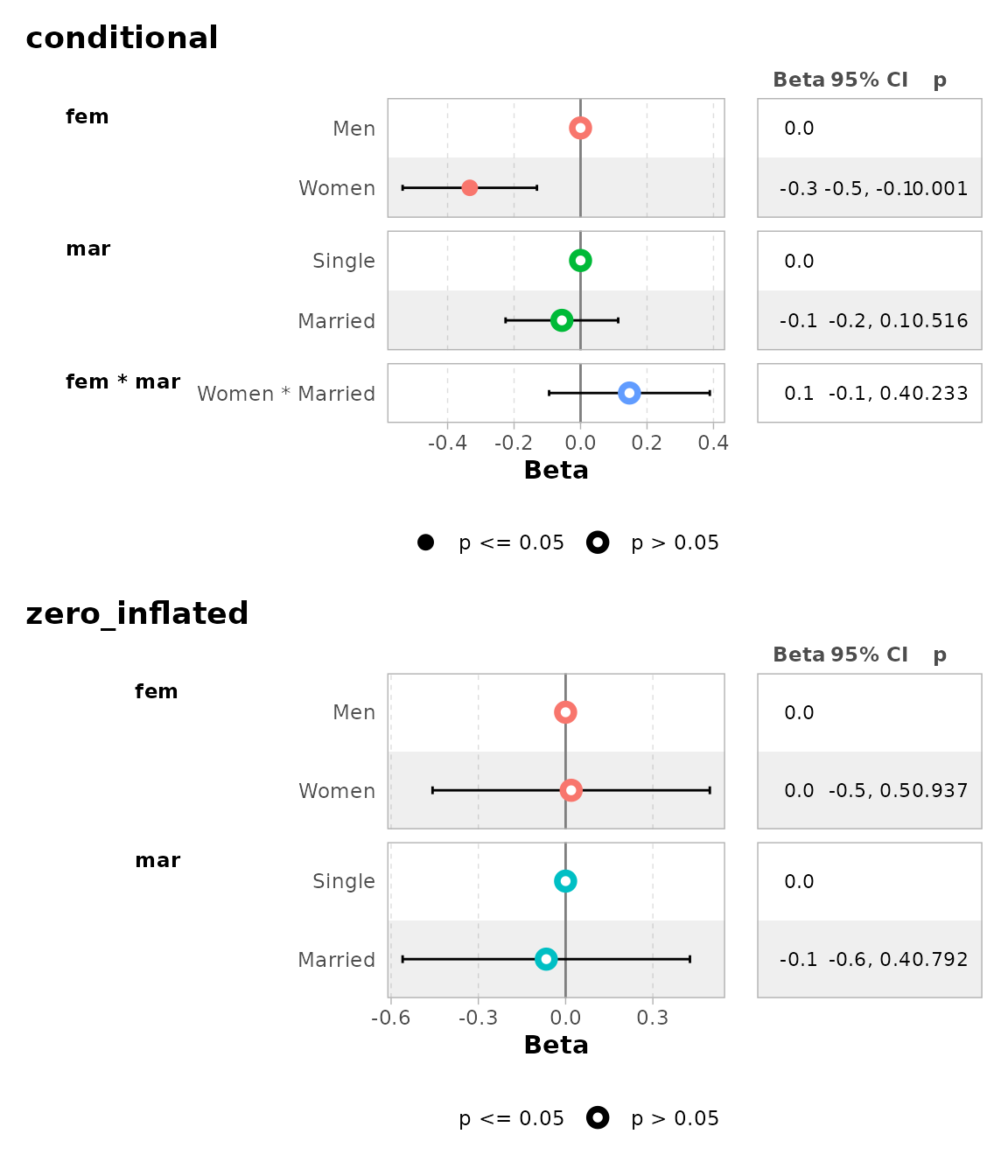ggcoef_compare(models, type = "faceted")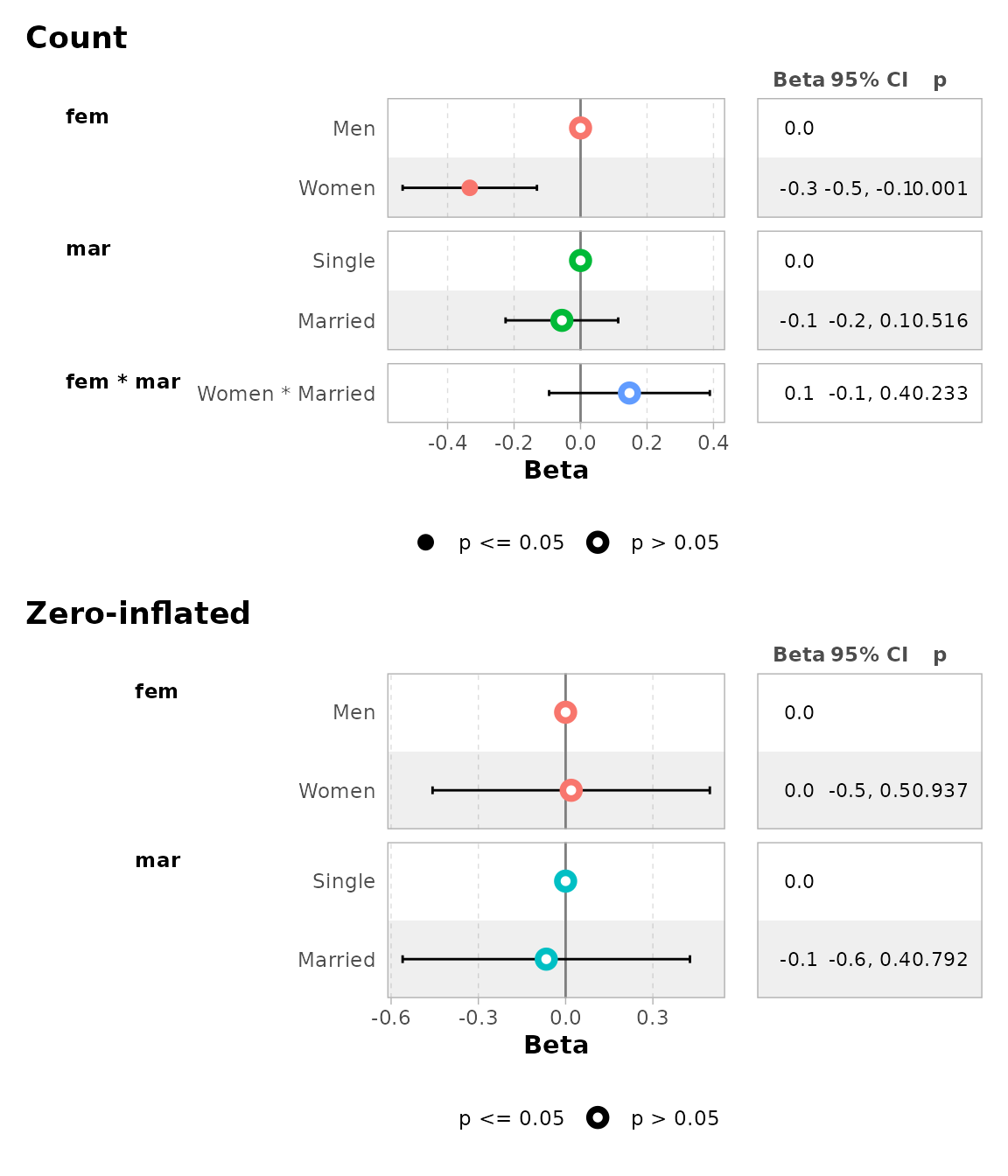Advanced users could use their own dataset and pass it to ggcoef_plot(). Such dataset could be produced by ggcoef_model(), ggcoef_compare() or ggcoef_multinom() with the option return_data = TRUE or by using broom::tidy() or broom.helpers::tidy_plus_plus().
betareg::betareg() Use tidy_parameters() as tidy_fun with component argument to control with coefficients to return. broom::tidy() does not support theexponentiateargument for betareg models, usetidy_parameters() instead.| |biglm::bigglm()| | |biglmm::bigglm()| | |brms::brm()|broom.mixedpackage required | |cmprsk::crr()|Limited support. It is recommended to usetidycmprsk::crr()instead. | |fixest::feglm()|May fail with R <= 4.0. | |fixest::femlm()|May fail with R <= 4.0. | |fixest::feNmlm()|May fail with R <= 4.0. | |fixest::feols()|May fail with R <= 4.0. | |gam::gam()| | |geepack::geeglm()| | |glmmTMB::glmmTMB()|broom.mixedpackage required | |lavaan::lavaan()|Limited support for categorical variables | |lfe::felm()| | |lme4::glmer.nb()|broom.mixedpackage required | |lme4::glmer()|broom.mixedpackage required | |lme4::lmer()|broom.mixedpackage required | |logitr::logitr()|Requires logitr >= 0.8.0 | |MASS::glm.nb()| | |MASS::polr()| | |mgcv::gam()|Use default tidierbroom::tidy()for smooth terms only, orgtsummary::tidy_gam()to include parametric terms | |mice::mira|Limited support. Ifmodis amiraobject, usetidy_plus_plus(mod, tidy_fun = function(x, …) mice::pool(x) %>% mice::tidy(…))| |multgee::nomLORgee()|Experimental support. Usetidy_multgee()astidy_fun. | |multgee::ordLORgee()|Experimental support. Usetidy_multgee()astidy_fun. | |nnet::multinom()| | |ordinal::clm()|Limited support for models with nominal predictors. | |ordinal::clmm()|Limited support for models with nominal predictors. | |parsnip::model_fit|Supported as long as the type of model and the engine is supported. | |plm::plm()| | |pscl::hurdle()|Usetidy_zeroinfl()astidy_fun. | |pscl::zeroinfl()|Usetidy_zeroinfl()astidy_fun. | |rstanarm::stan_glm()|broom.mixedpackage required | |stats::aov()|Reference rows are not relevant for such models. | |stats::glm()| | |stats::lm()| | |stats::nls()|Limited support | |survey::svycoxph()| | |survey::svyglm()| | |survey::svyolr()| | |survival::clogit()| | |survival::coxph()| | |survival::survreg()| | |tidycmprsk::crr()| | |VGAM::vglm()|Limited support. It is recommended to usetidy_parameters()astidy_fun.
Note: this list of models has been tested. broom.helpers, and therefore ggcoef_model()`, may or may not work properly or partially with other types of models.